# RD Sharma Solutions for Class 9 Maths Chapter 10 Congruent Triangles

RD Sharma Solutions for Class 9 Chapter 10 are provided here. In this chapter, students will study about a fundamental concept of Geometry known as “Congruence”. This concept is used to classify geometrical figures on the basis of their shapes. Two geometrical figures are said to be congruent if they have exactly the same size and same shape.

Rules for congruence of triangles are as follows:

SSS (Side-Side-Side): If all the sides of two triangles are equivalent, then triangles are said to be congruent.

SAS (Side-Angle-Side): If two sides and an interior angle of a triangle are equal to the corresponding sides and an interior angle of the other triangle, then both triangles are said to be congruent.

ASA (Angle-Side-Angle): If two angles and included side of one triangle are equal to the corresponding two angles and included side of another triangle, then the triangles are congruent.

RHS (Right angle- Hypotenuse-Side): If in two right-angled triangles, the hypotenuse and any one side of a triangle are equivalent to the hypotenuse and one side of the other triangle, then both triangles are said to be congruent.

Check the detailed RD Sharma Class 9 solutions for all chapters and start practicing to score good marks.

## Download PDF of RD Sharma Solutions for Class 9 Chapter 10 Congruent Triangles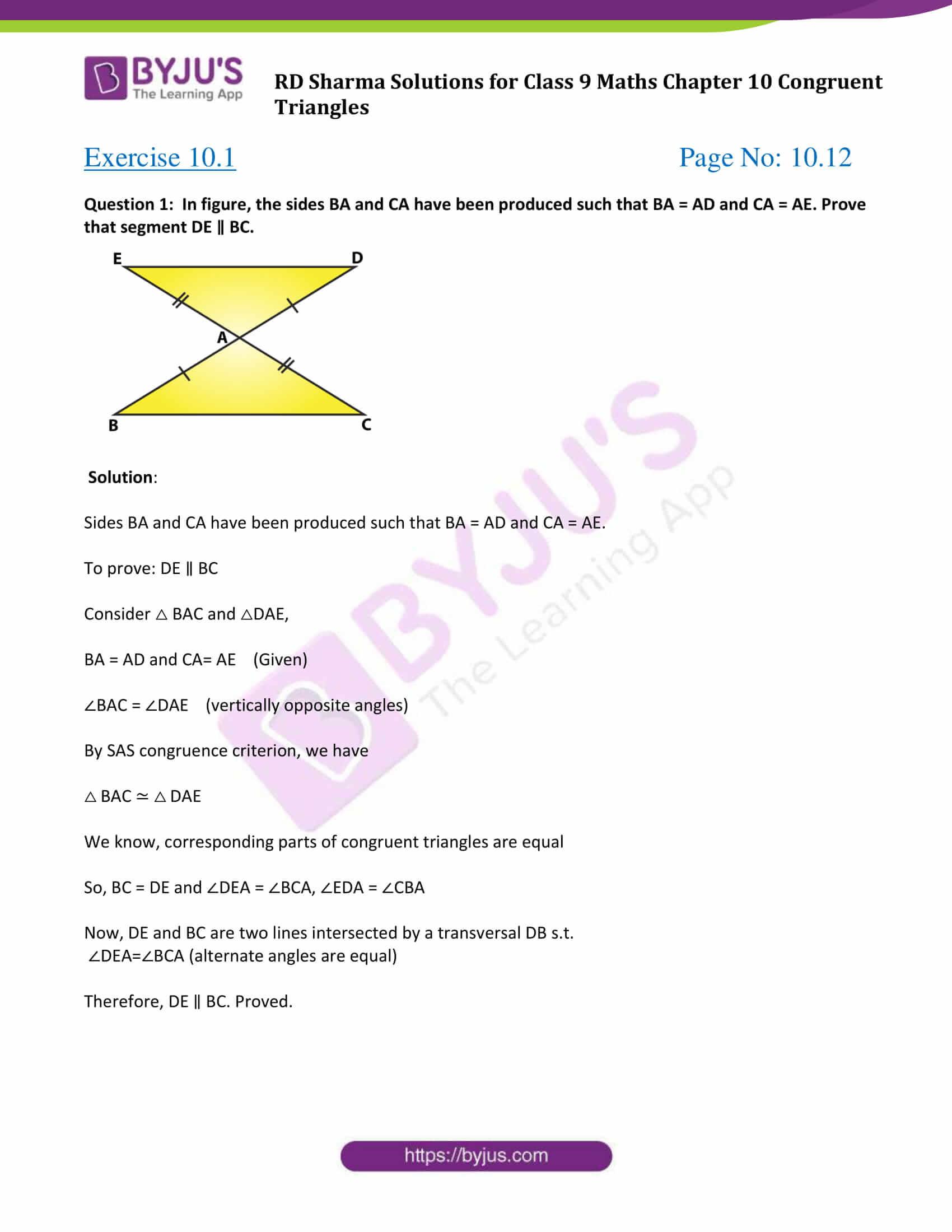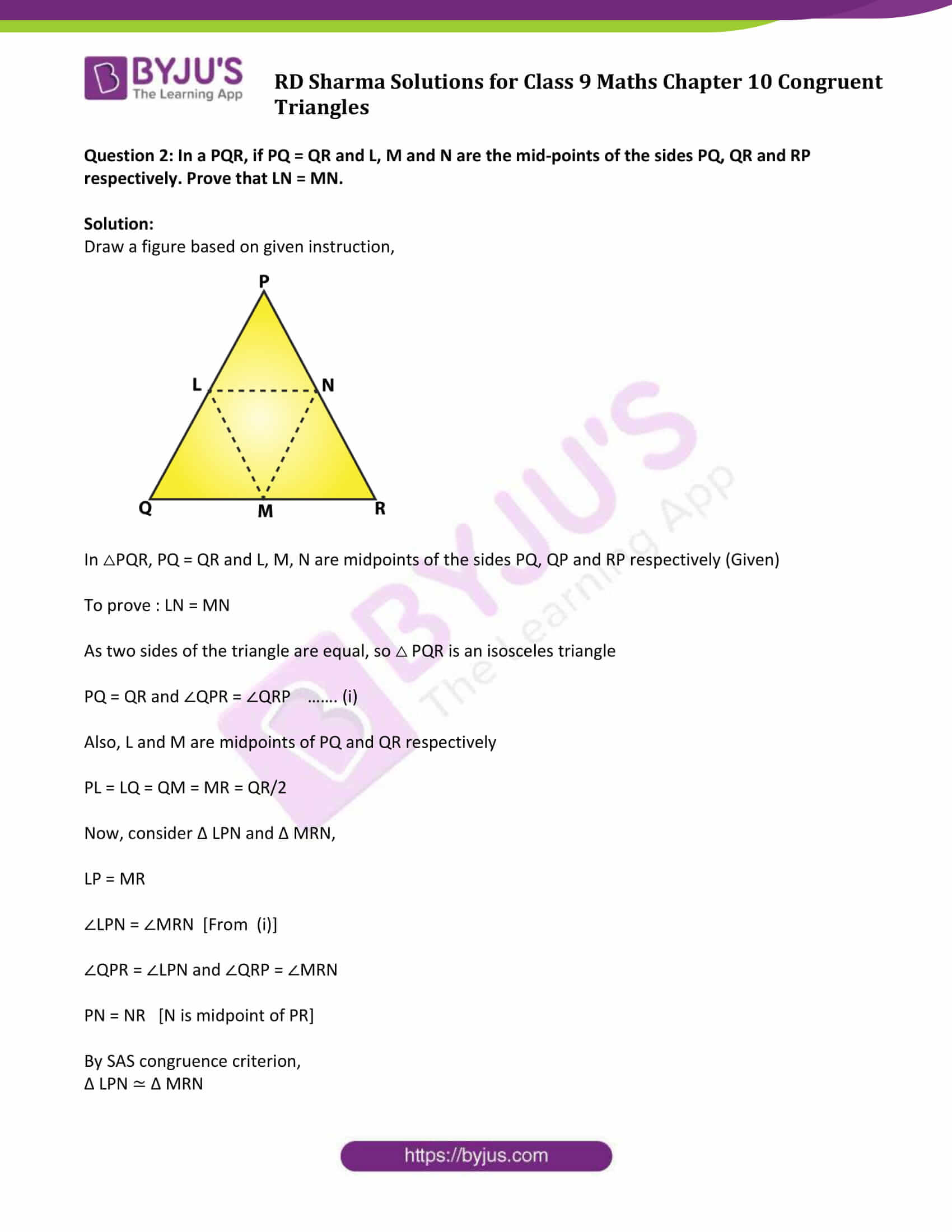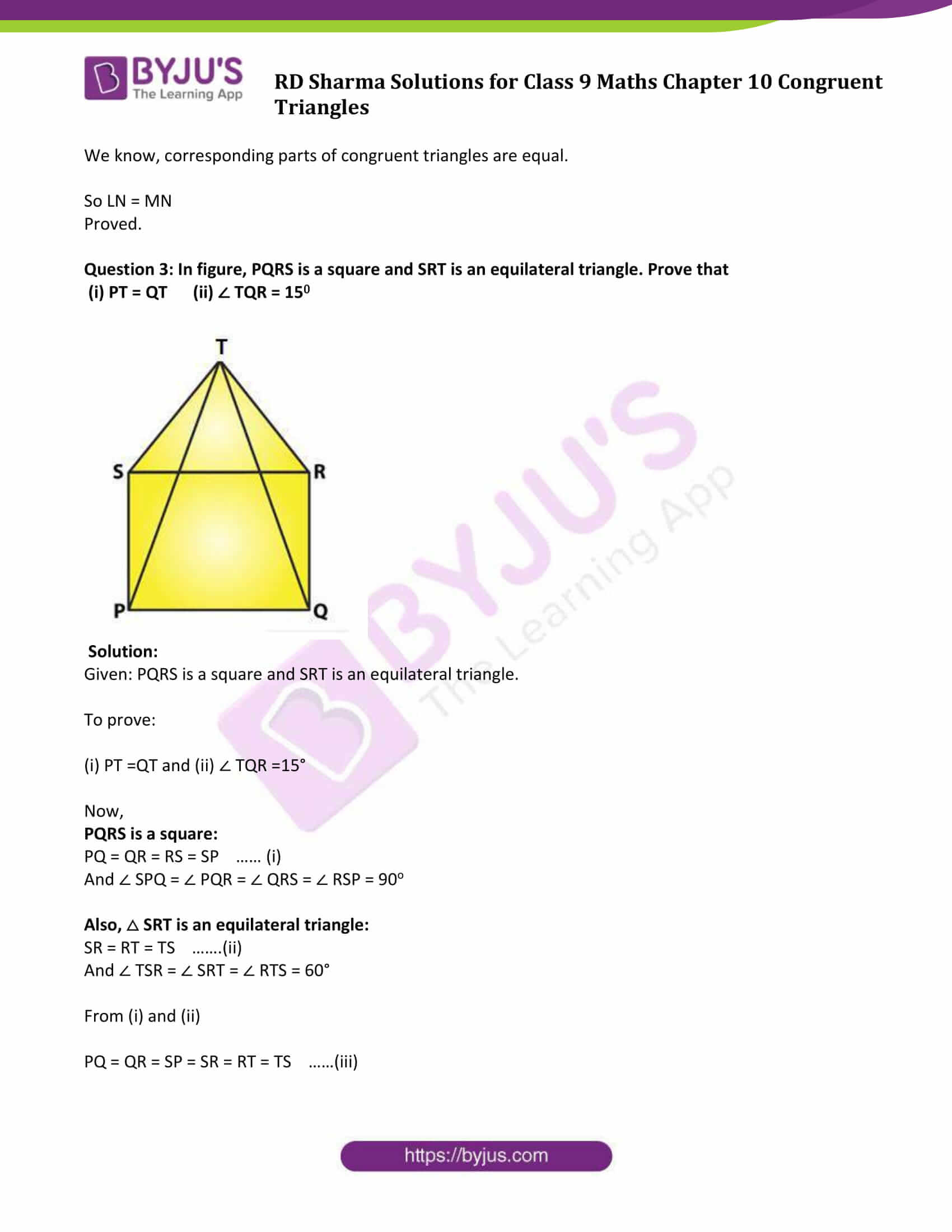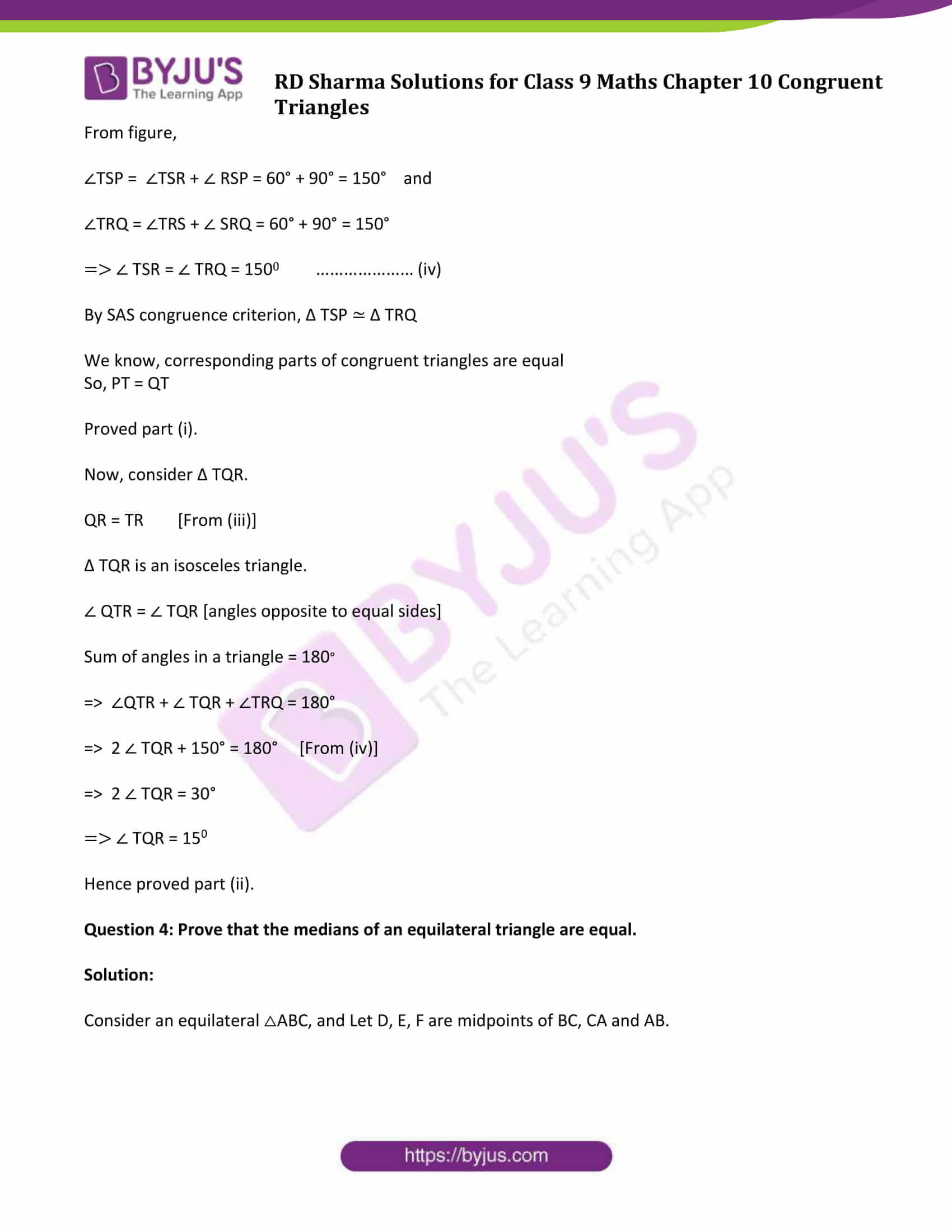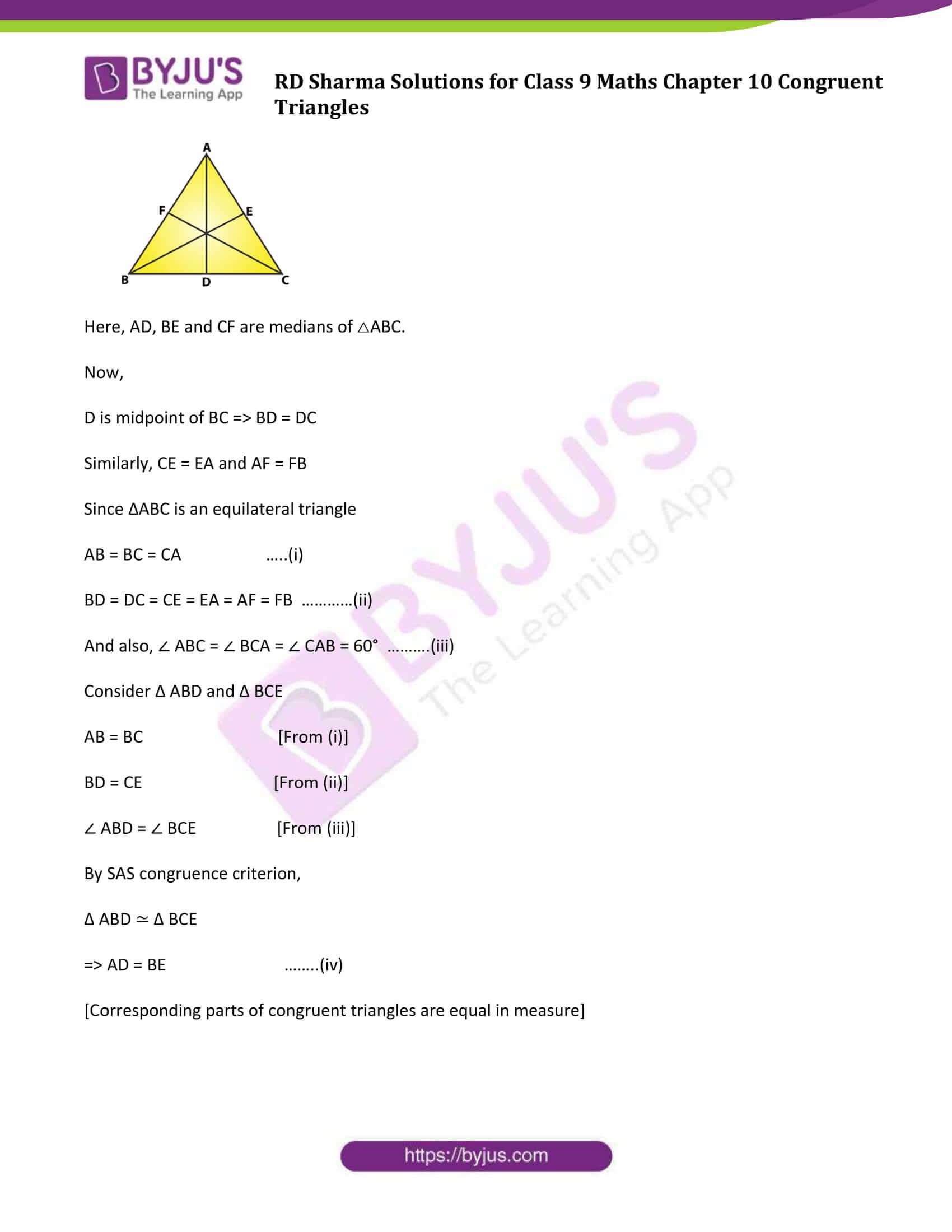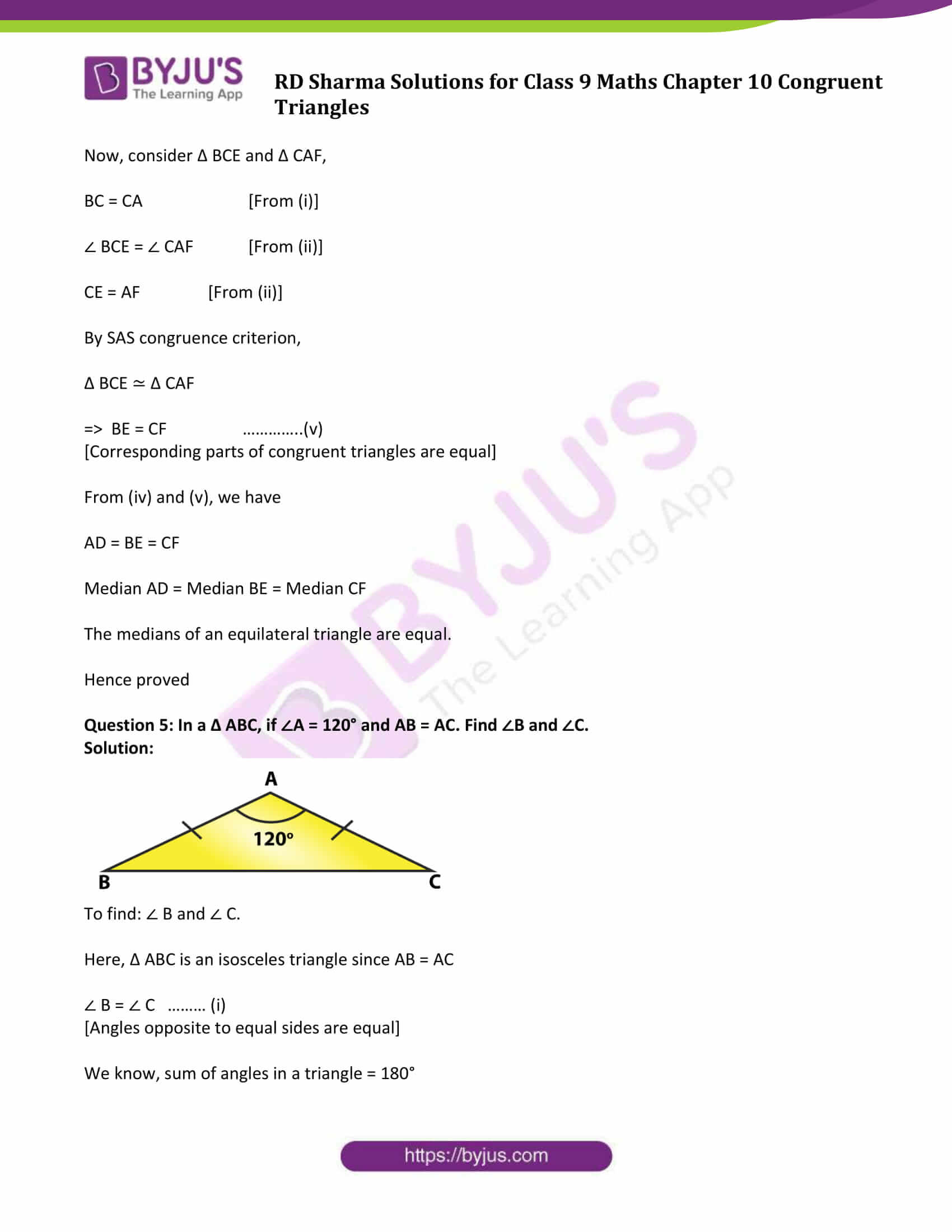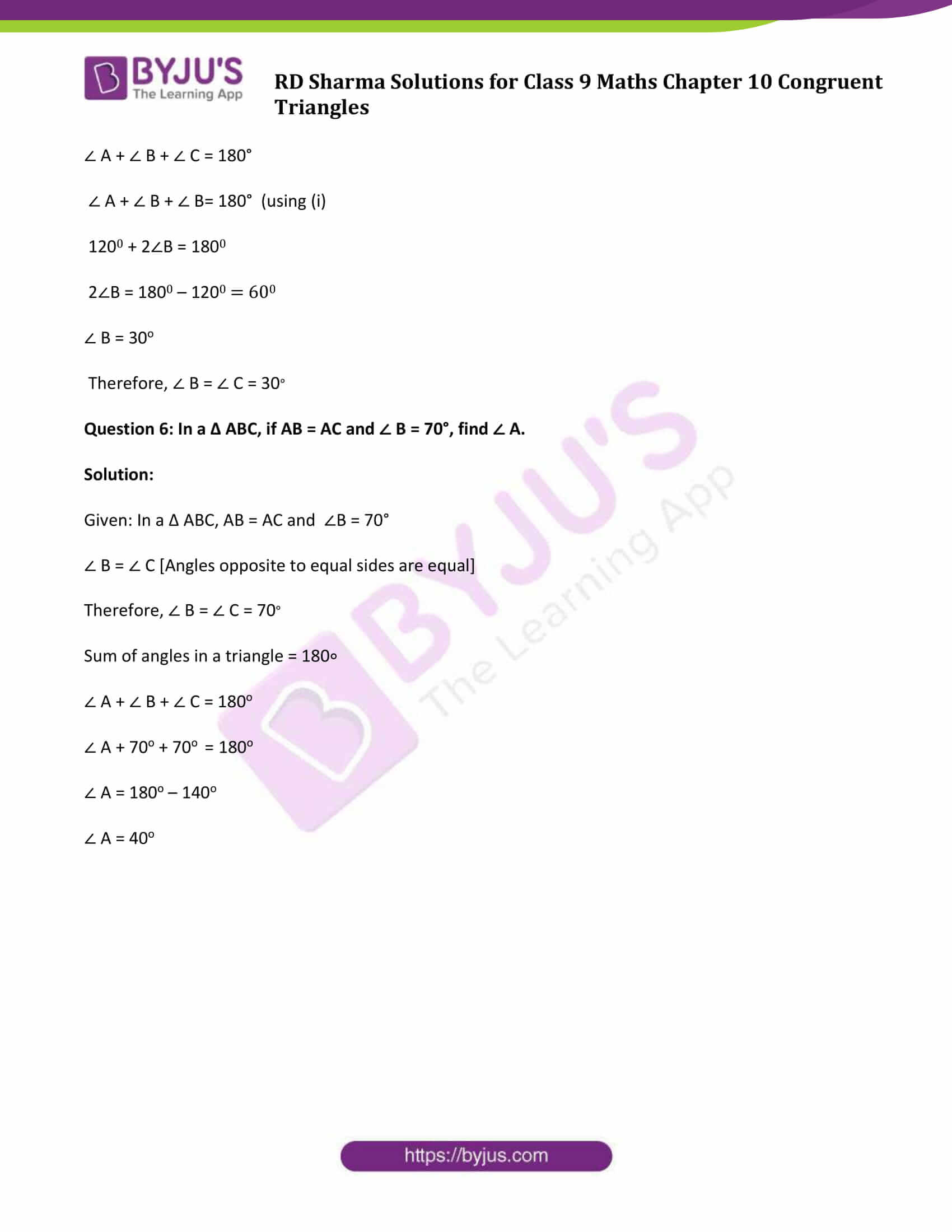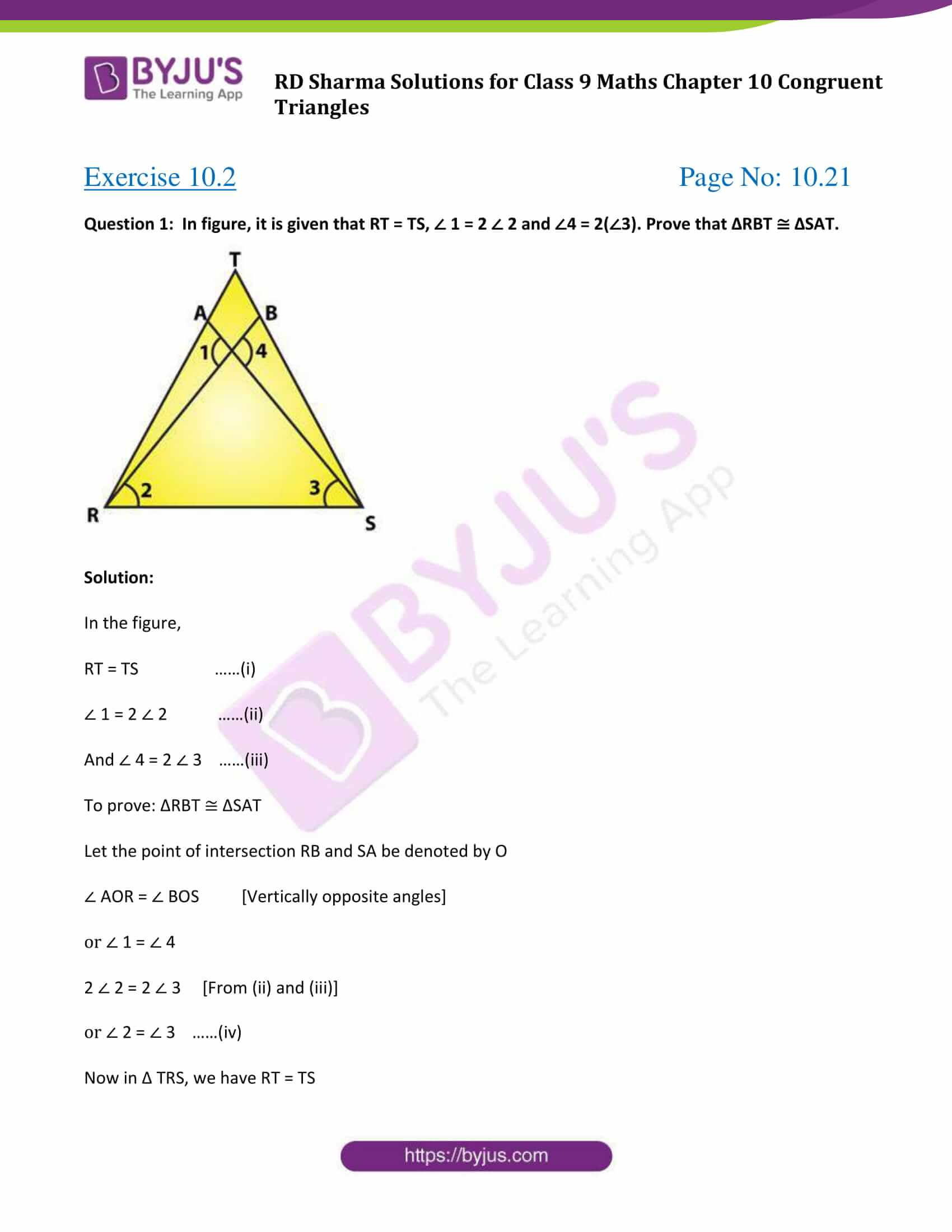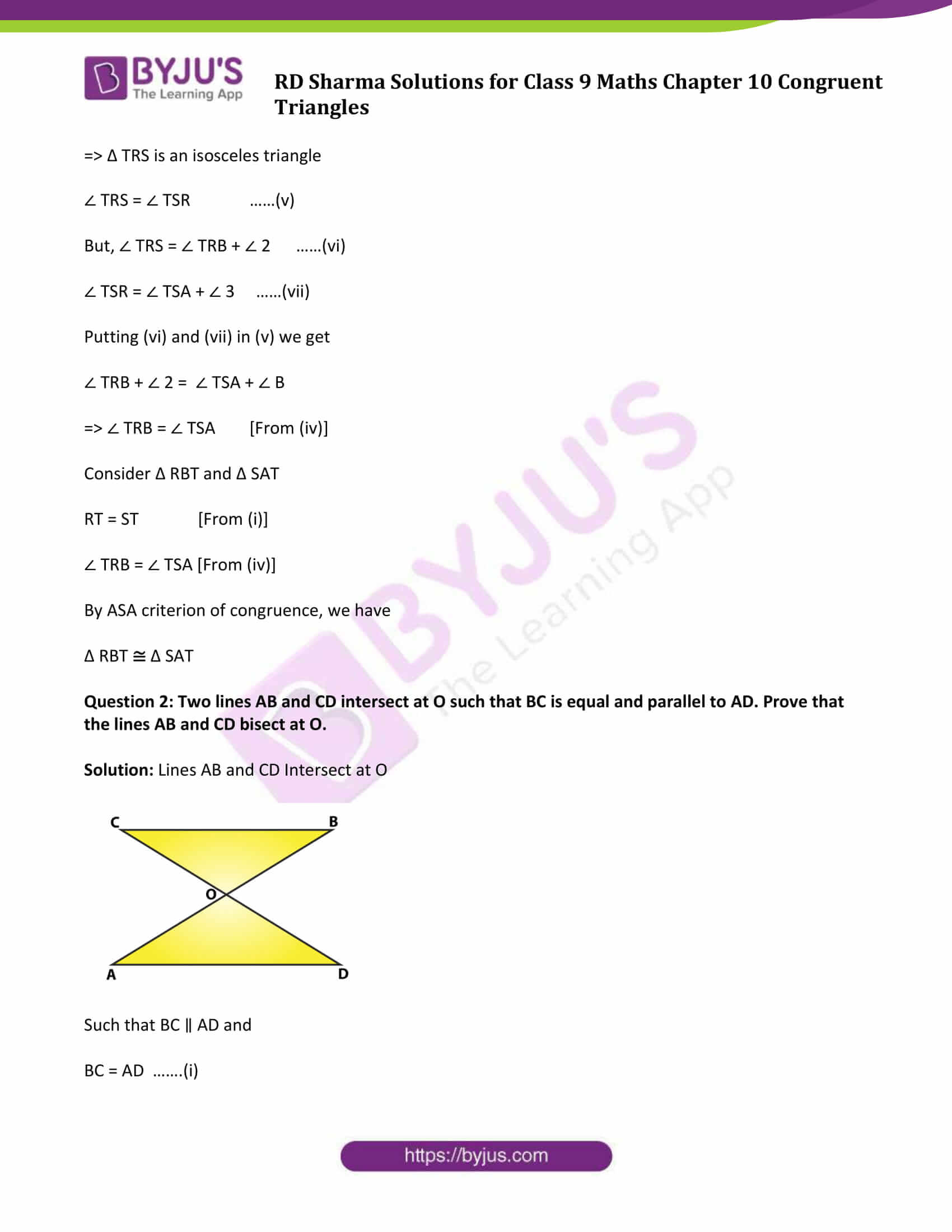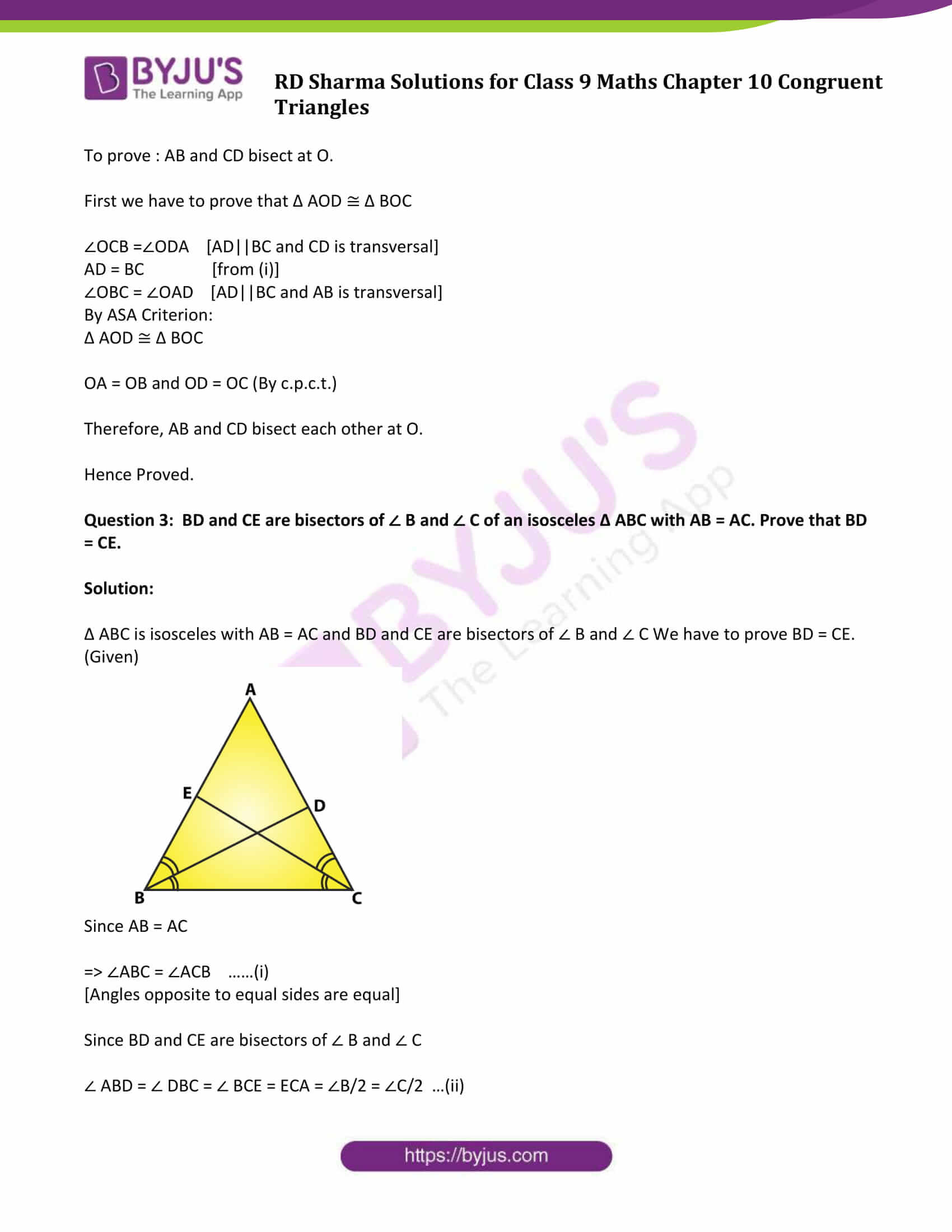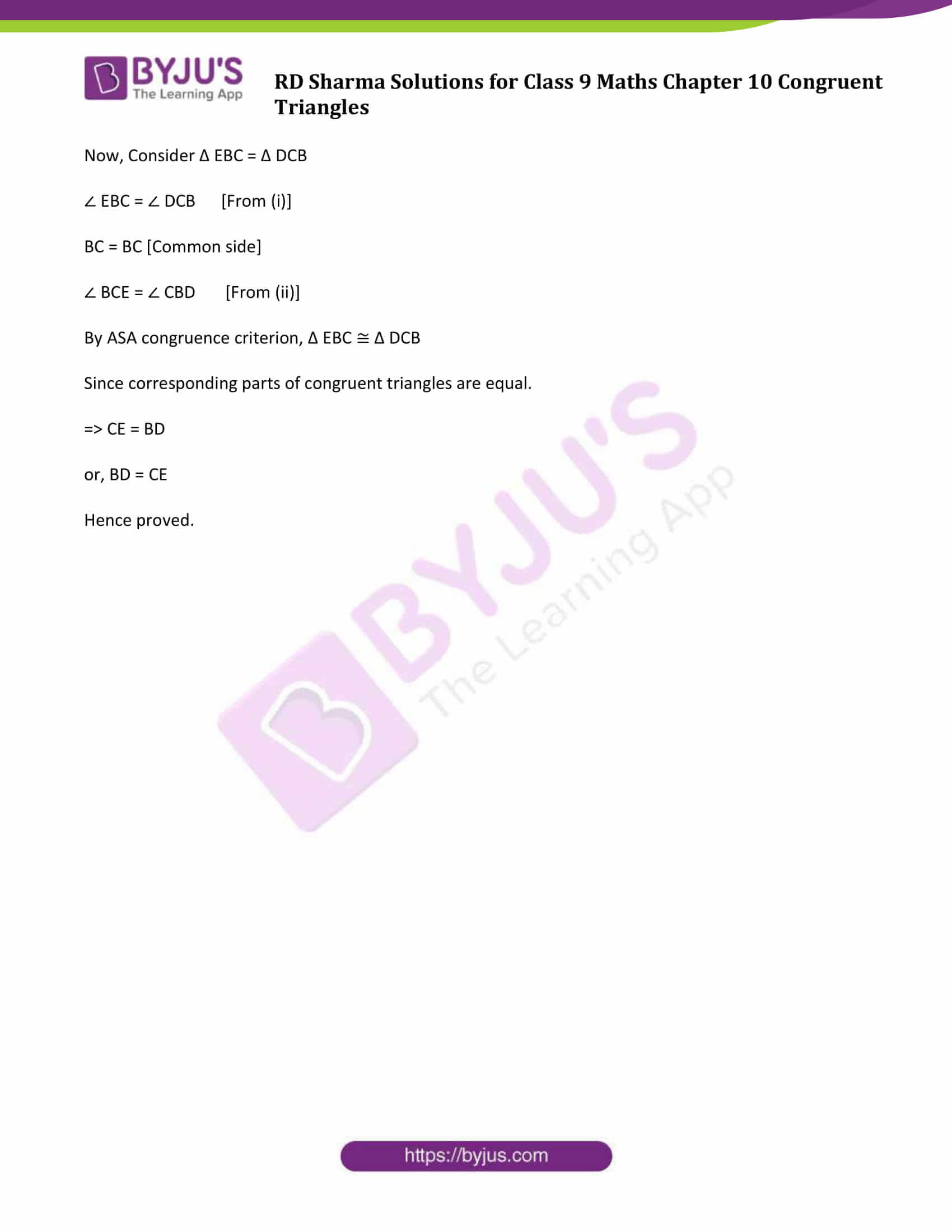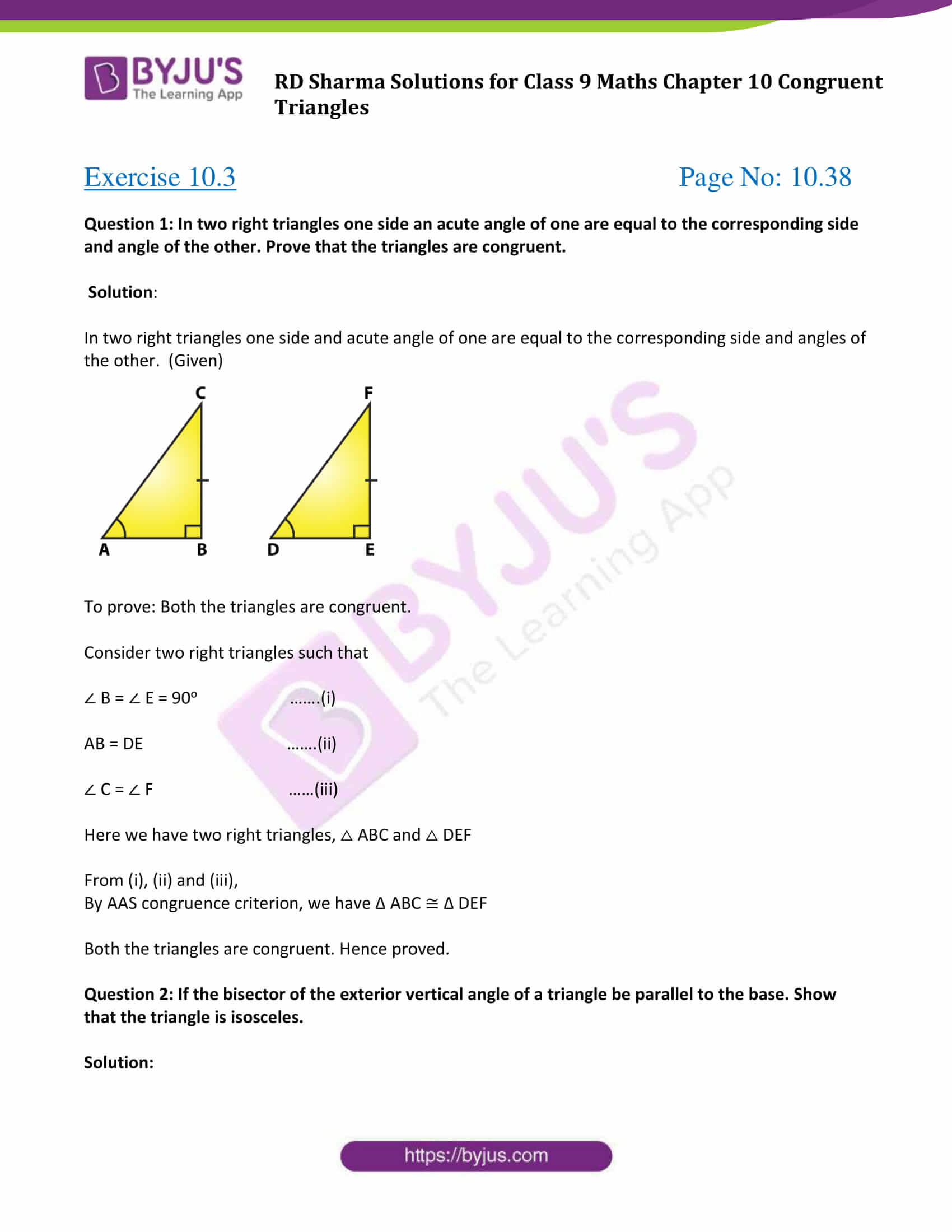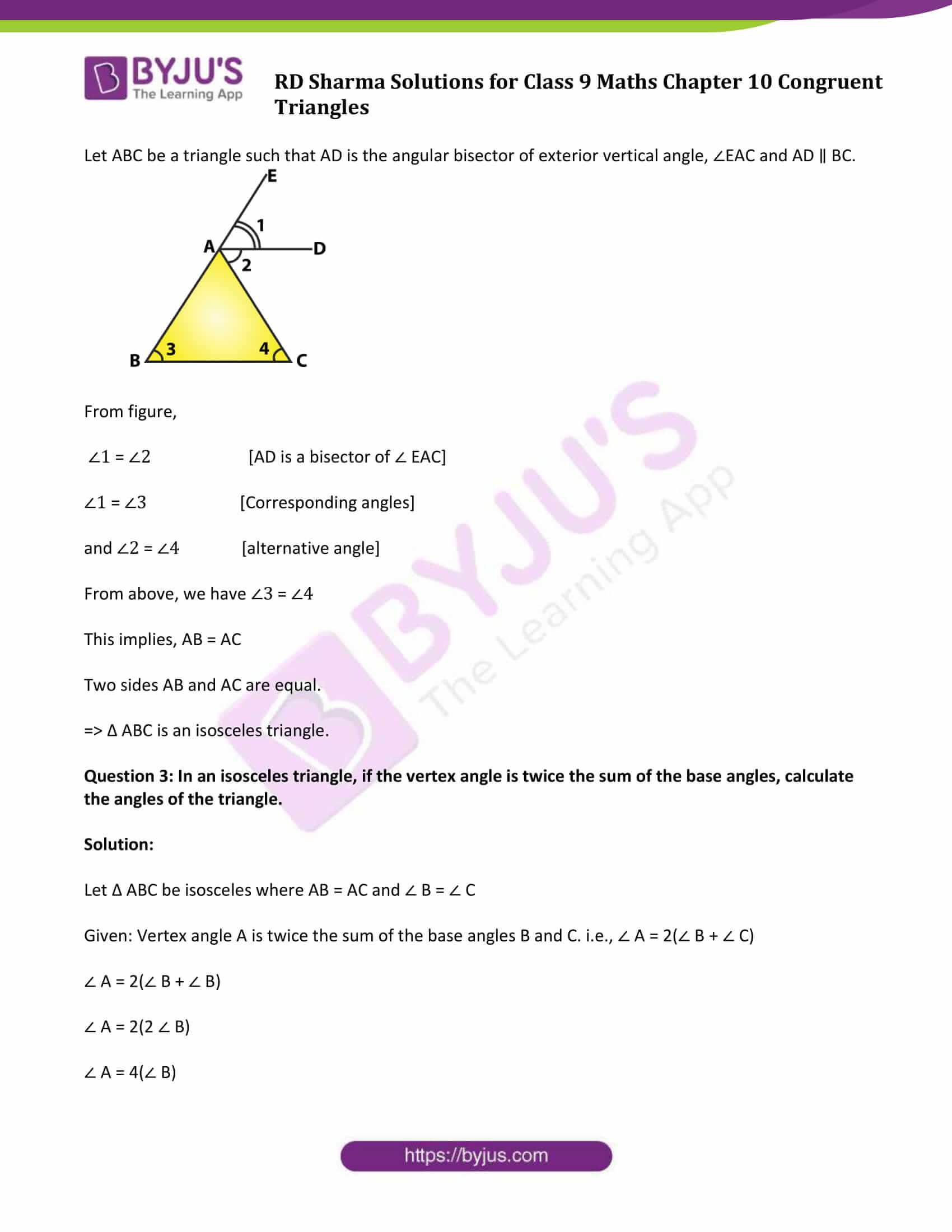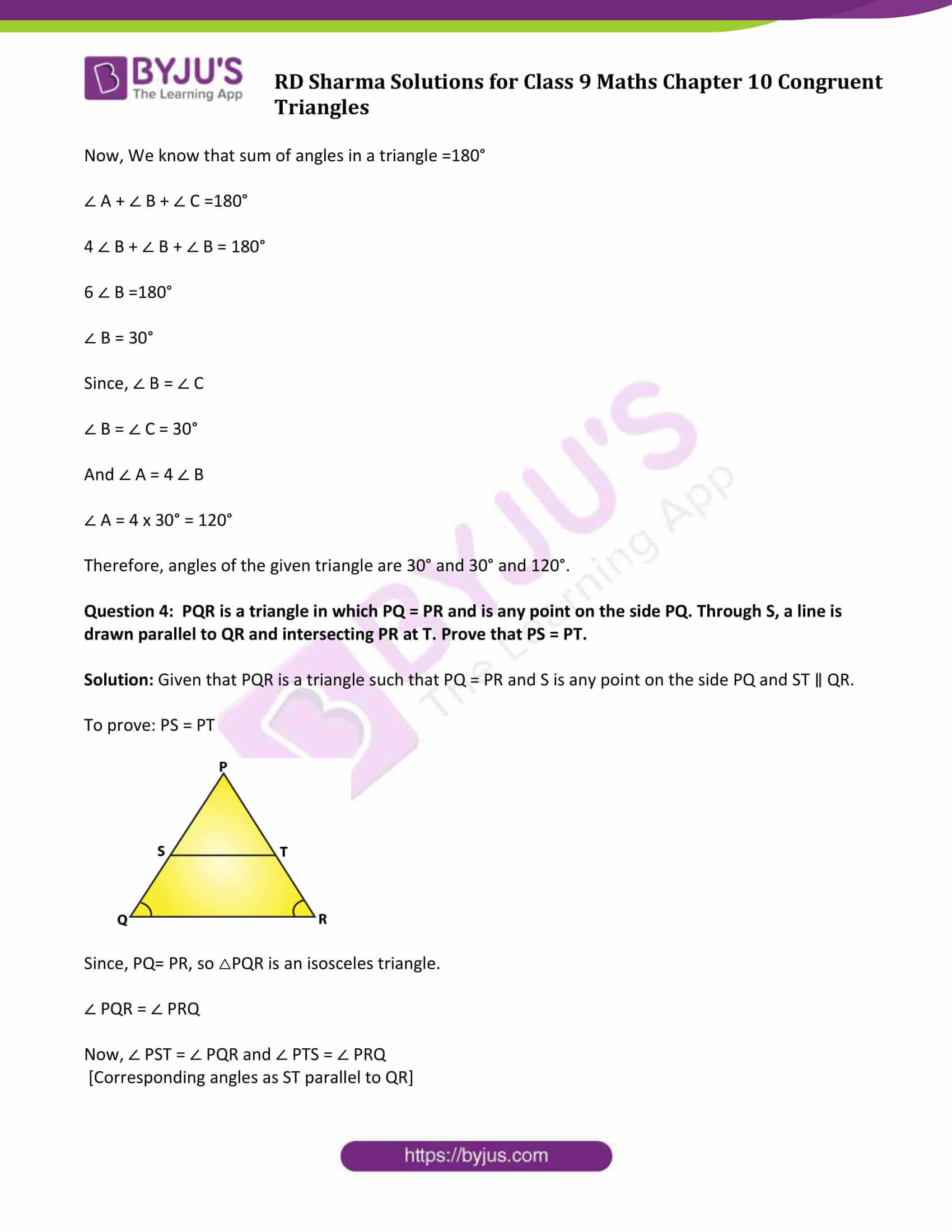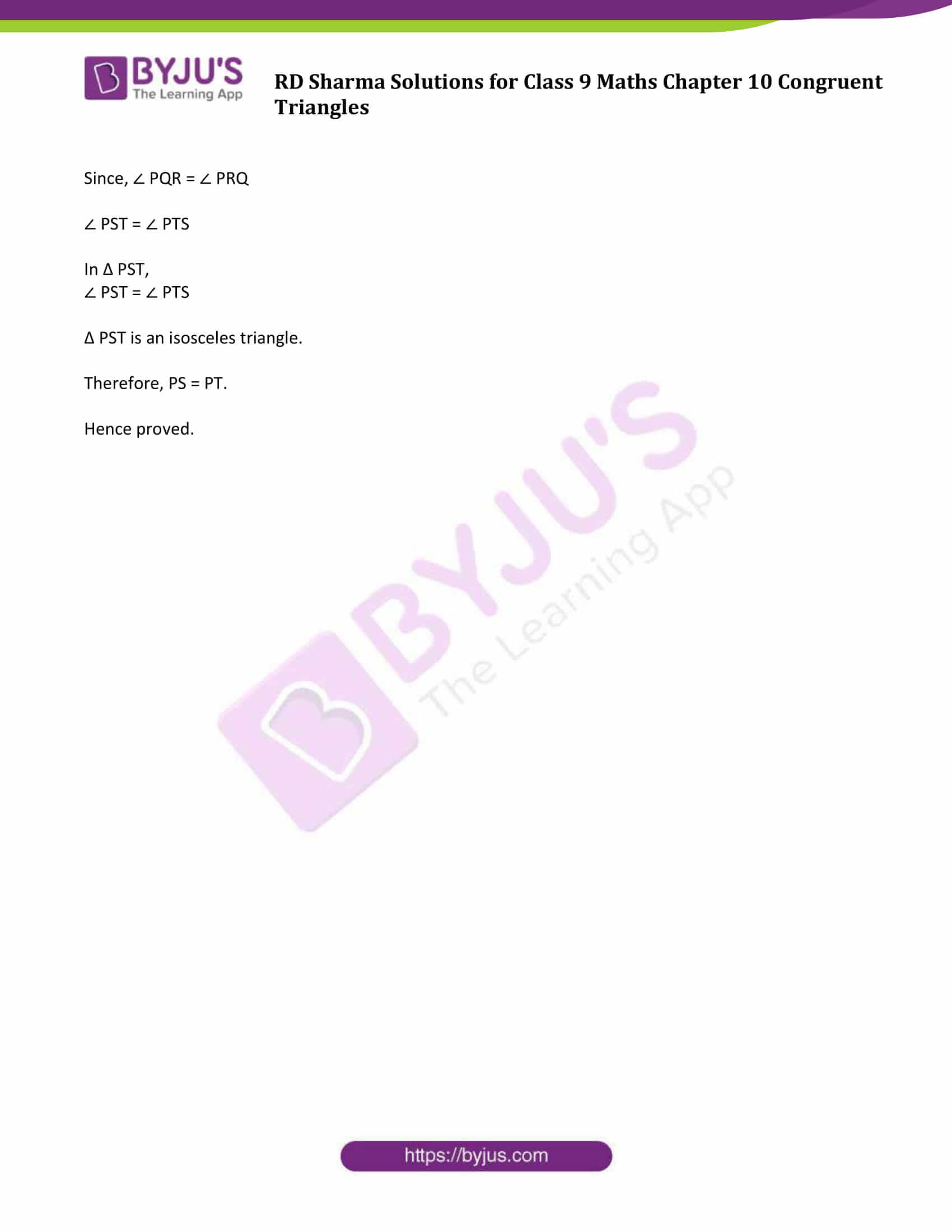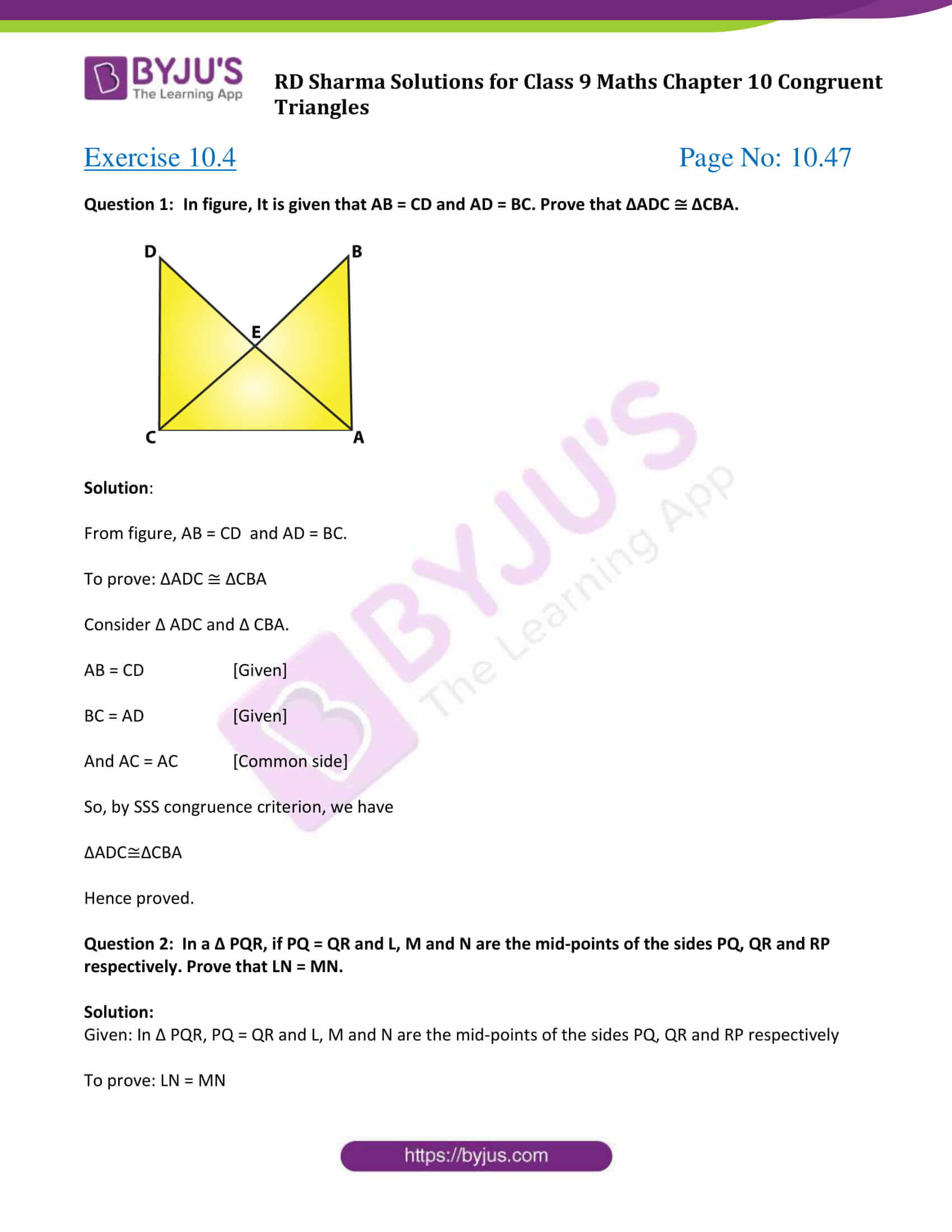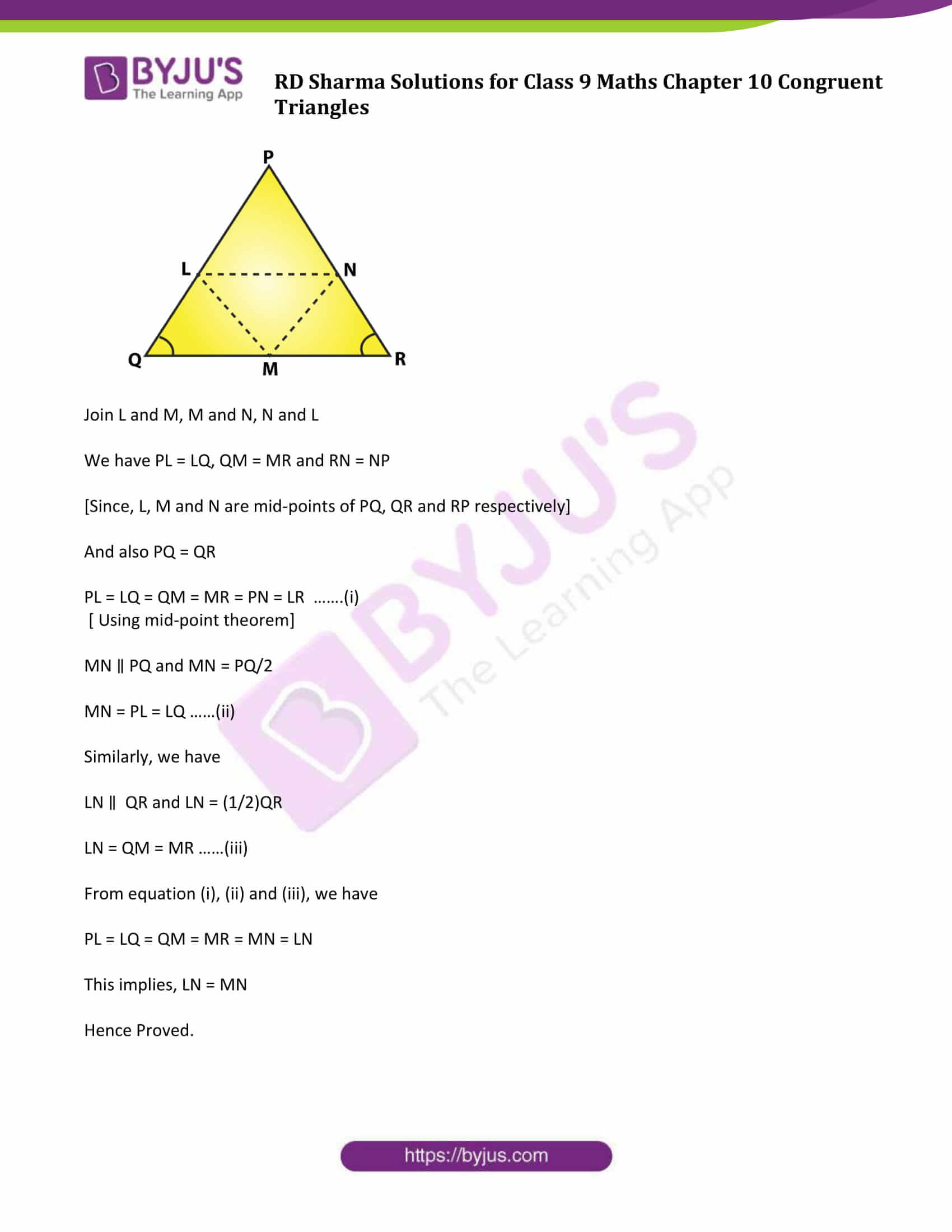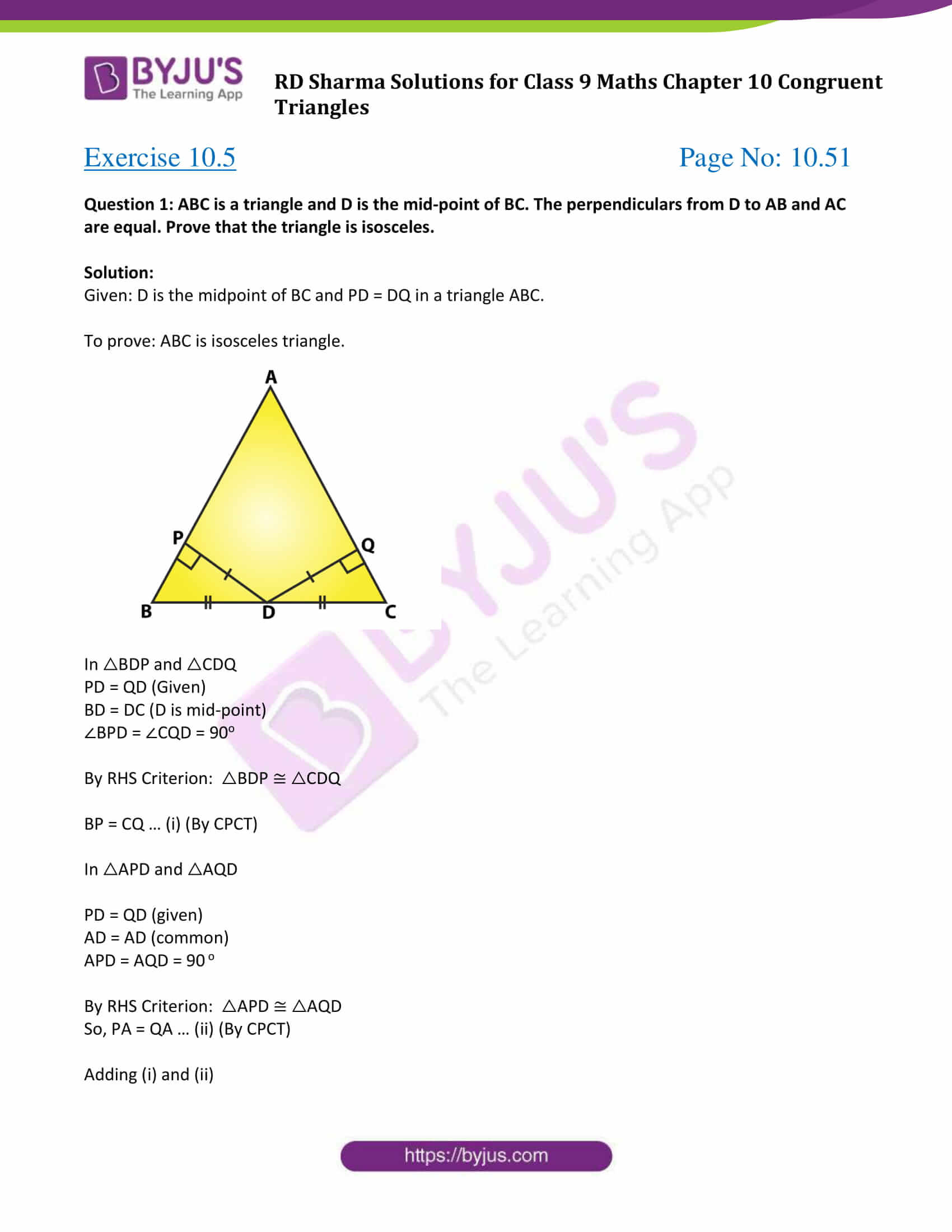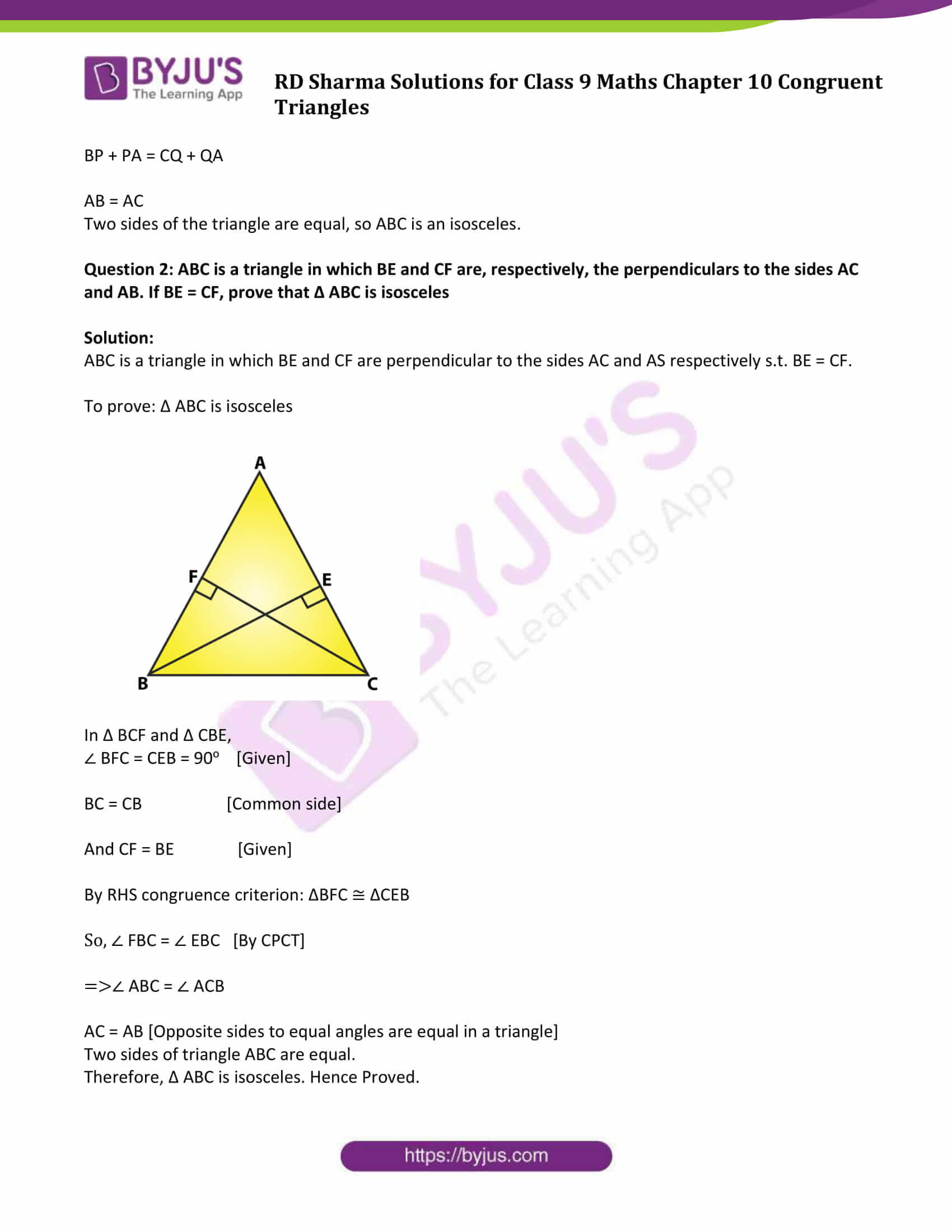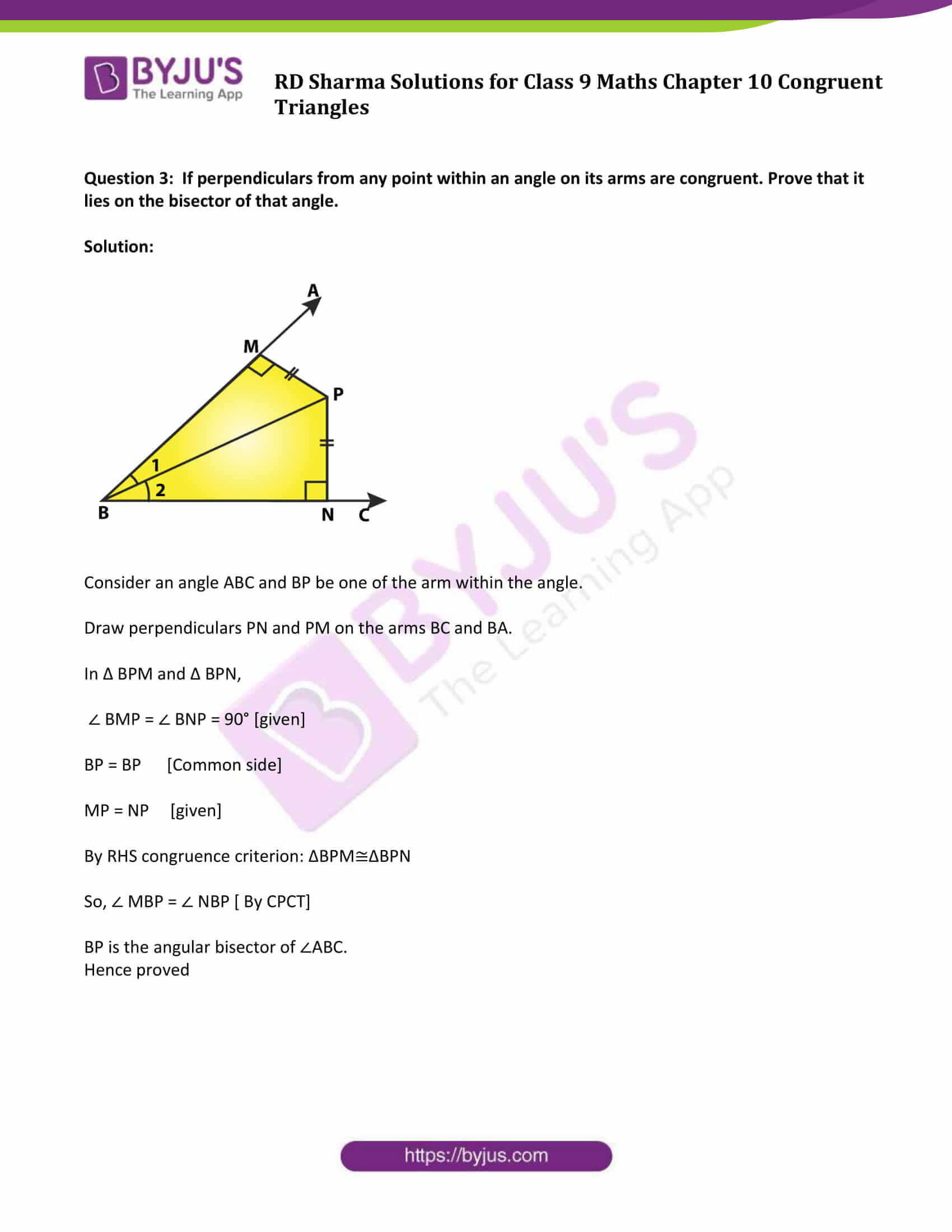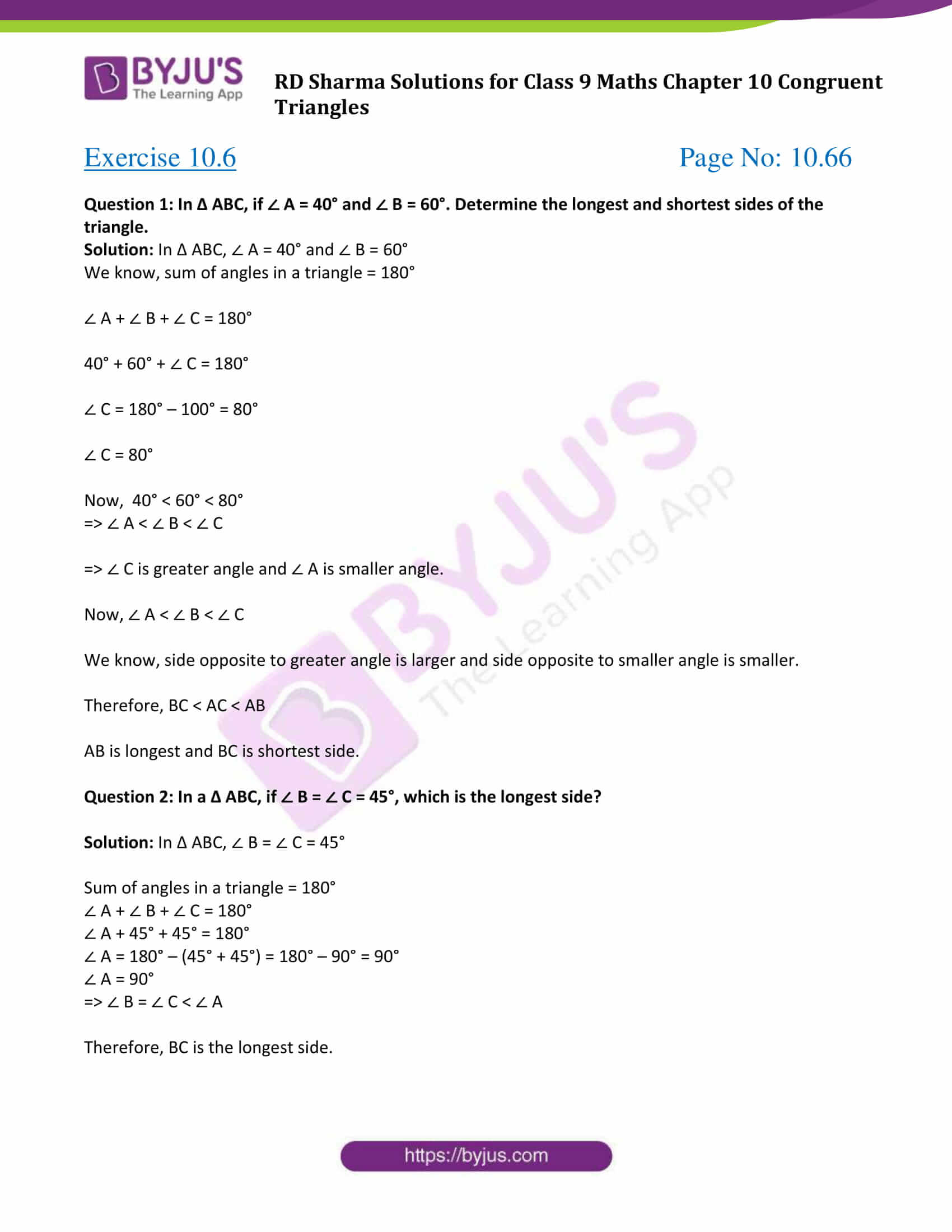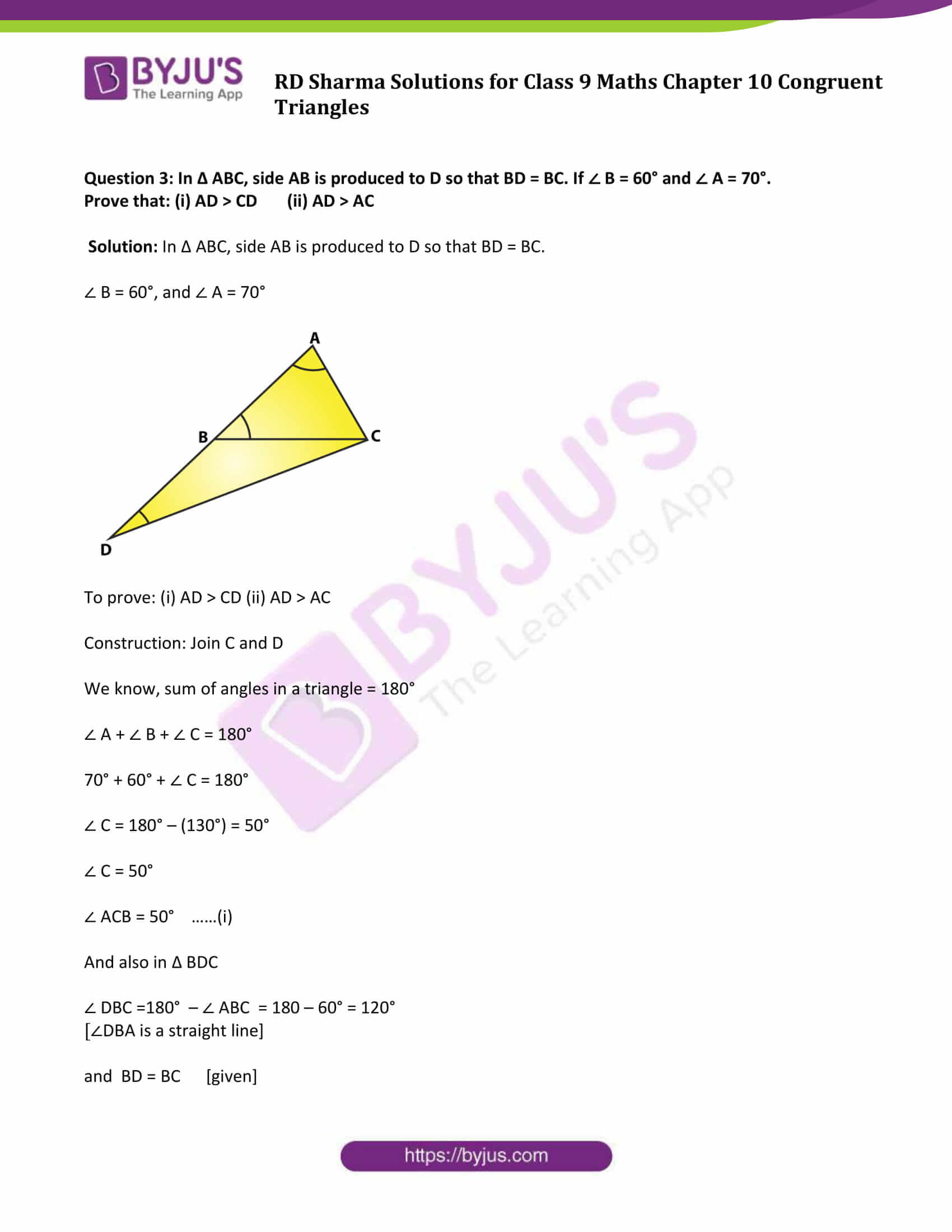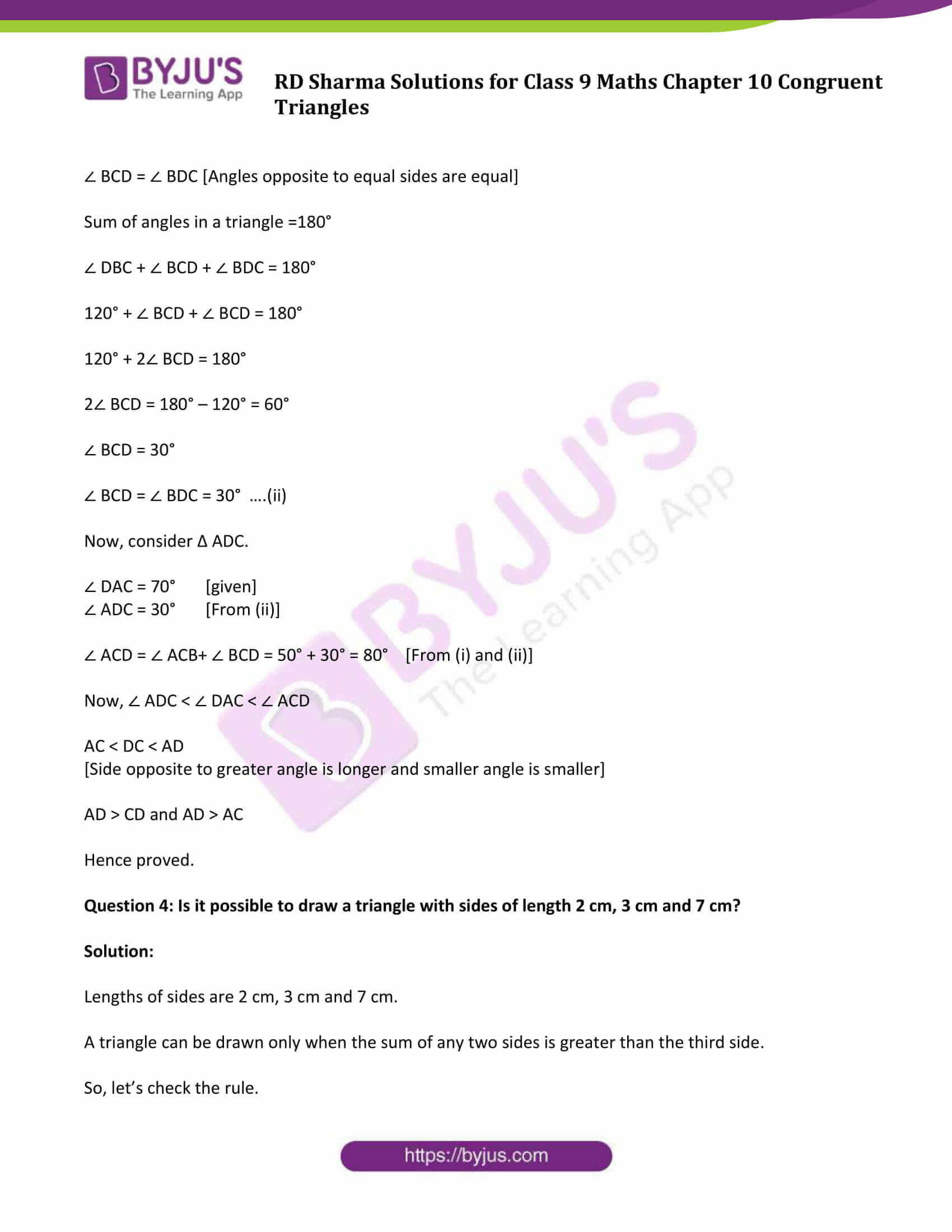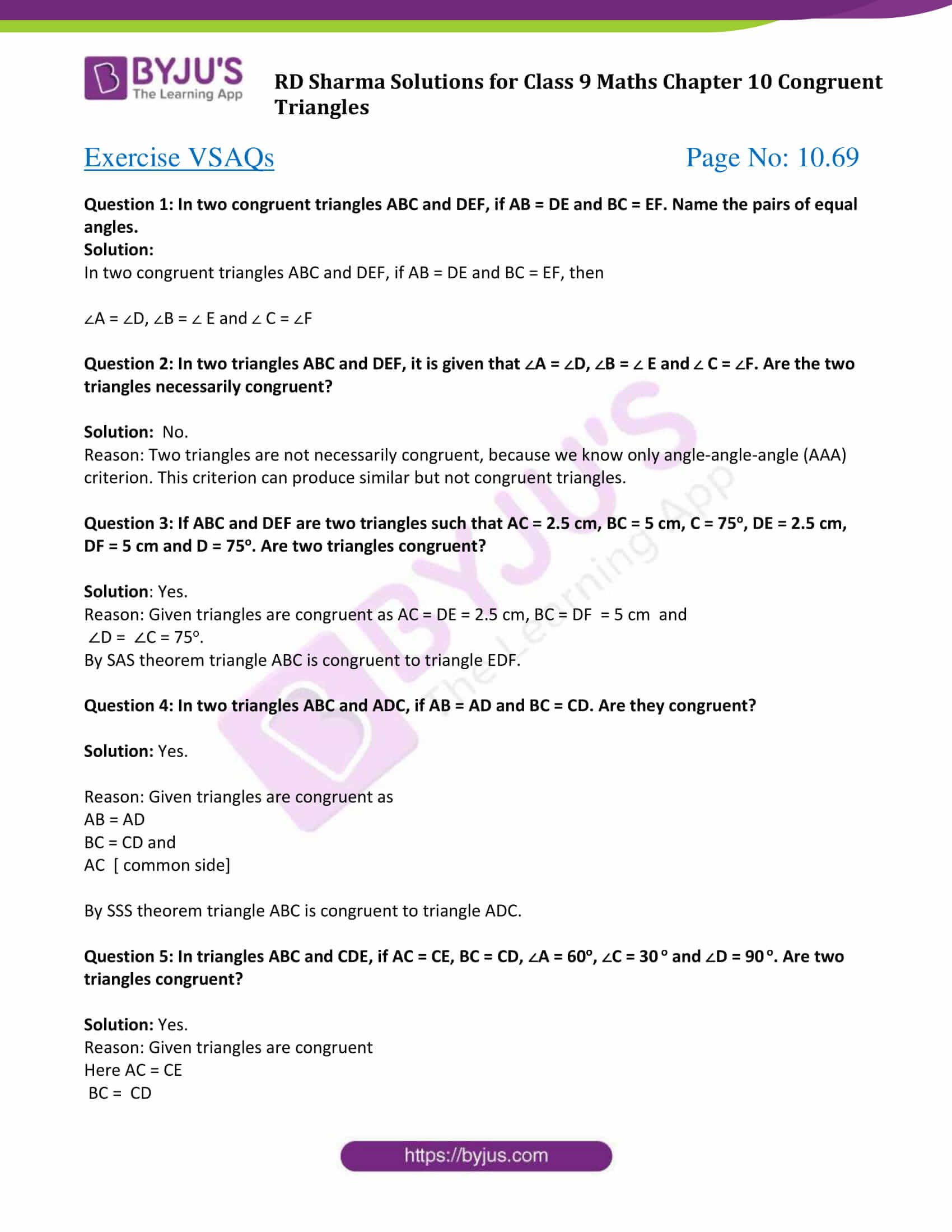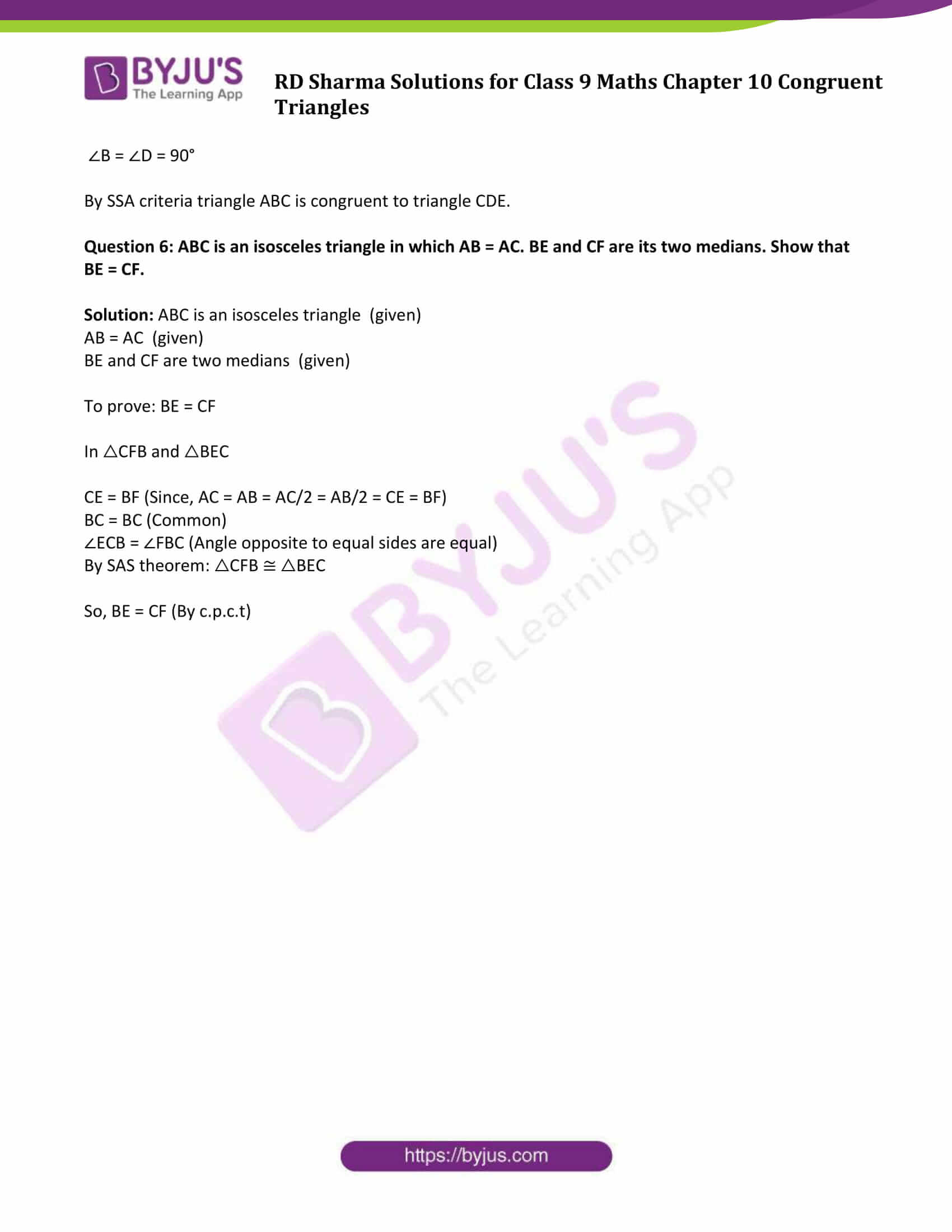### Exercise 10.1

Question 1: In figure, the sides BA and CA have been produced such that BA = AD and CA = AE. Prove that segment DE ∥ BC.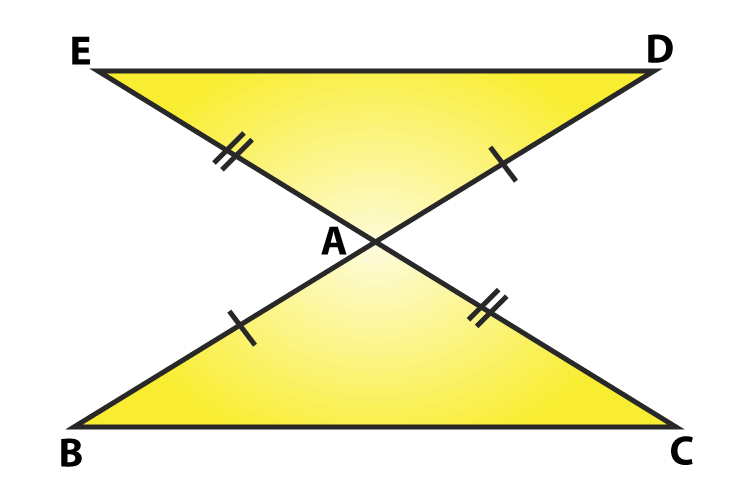Solution:

Sides BA and CA have been produced such that BA = AD and CA = AE.

To prove: DE ∥ BC

Consider △ BAC and △DAE,

BA = AD and CA= AE (Given)

∠BAC = ∠DAE (vertically opposite angles)

By SAS congruence criterion, we have

△ BAC ≃ △ DAE

We know, corresponding parts of congruent triangles are equal

So, BC = DE and ∠DEA = ∠BCA, ∠EDA = ∠CBA

Now, DE and BC are two lines intersected by a transversal DB s.t.

∠DEA=∠BCA (alternate angles are equal)

Therefore, DE ∥ BC. Proved.

Question 2: In a PQR, if PQ = QR and L, M and N are the mid-points of the sides PQ, QR and RP respectively. Prove that LN = MN.

Solution:

Draw a figure based on given instruction,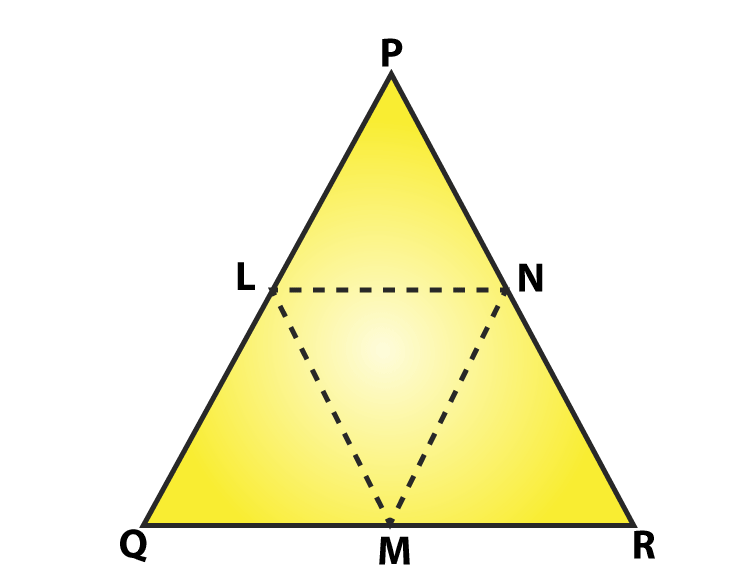In △PQR, PQ = QR and L, M, N are midpoints of the sides PQ, QP and RP respectively (Given)

To prove : LN = MN

As two sides of the triangle are equal, so △ PQR is an isosceles triangle

PQ = QR and ∠QPR = ∠QRP ……. (i)

Also, L and M are midpoints of PQ and QR respectively

PL = LQ = QM = MR = QR/2

Now, consider Δ LPN and Δ MRN,

LP = MR

∠LPN = ∠MRN [From (i)]

∠QPR = ∠LPN and ∠QRP = ∠MRN

PN = NR [N is midpoint of PR]

By SAS congruence criterion,

Δ LPN ≃ Δ MRN

We know, corresponding parts of congruent triangles are equal.

So LN = MN

Proved.

Question 3: In figure, PQRS is a square and SRT is an equilateral triangle. Prove that

(i) PT = QT (ii) ∠ TQR = 150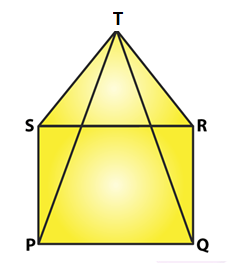Solution:

Given: PQRS is a square and SRT is an equilateral triangle.

To prove:

(i) PT =QT and (ii) ∠ TQR =15°

Now,

PQRS is a square:

PQ = QR = RS = SP …… (i)

And ∠ SPQ = ∠ PQR = ∠ QRS = ∠ RSP = 90o

Also, △ SRT is an equilateral triangle:

SR = RT = TS …….(ii)

And ∠ TSR = ∠ SRT = ∠ RTS = 60°

From (i) and (ii)

PQ = QR = SP = SR = RT = TS ……(iii)

From figure,

∠TSP = ∠TSR + ∠ RSP = 60° + 90° = 150° and

∠TRQ = ∠TRS + ∠ SRQ = 60° + 90° = 150°

=> ∠ TSR = ∠ TRQ = 1500 ………………… (iv)

By SAS congruence criterion, Δ TSP ≃ Δ TRQ

We know, corresponding parts of congruent triangles are equal

So, PT = QT

Proved part (i).

Now, consider Δ TQR.

QR = TR [From (iii)]

Δ TQR is an isosceles triangle.

∠ QTR = ∠ TQR [angles opposite to equal sides]

Sum of angles in a triangle = 180

=> ∠QTR + ∠ TQR + ∠TRQ = 180°

=> 2 ∠ TQR + 150° = 180° [From (iv)]

=> 2 ∠ TQR = 30°

=> ∠ TQR = 150

Hence proved part (ii).

Question 4: Prove that the medians of an equilateral triangle are equal.

Solution:

Consider an equilateral △ABC, and Let D, E, F are midpoints of BC, CA and AB.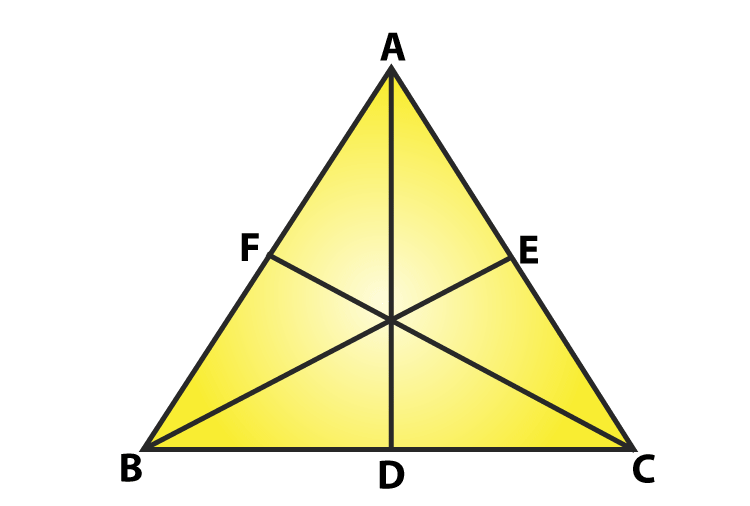Here, AD, BE and CF are medians of △ABC.

Now,

D is midpoint of BC => BD = DC

Similarly, CE = EA and AF = FB

Since ΔABC is an equilateral triangle

AB = BC = CA …..(i)

BD = DC = CE = EA = AF = FB …………(ii)

And also, ∠ ABC = ∠ BCA = ∠ CAB = 60° ……….(iii)

Consider Δ ABD and Δ BCE

AB = BC [From (i)]

BD = CE [From (ii)]

∠ ABD = ∠ BCE [From (iii)]

By SAS congruence criterion,

Δ ABD ≃ Δ BCE

[Corresponding parts of congruent triangles are equal in measure]

Now, consider Δ BCE and Δ CAF,

BC = CA [From (i)]

∠ BCE = ∠ CAF [From (ii)]

CE = AF [From (ii)]

By SAS congruence criterion,

Δ BCE ≃ Δ CAF

=> BE = CF …………..(v)

[Corresponding parts of congruent triangles are equal]

From (iv) and (v), we have

Median AD = Median BE = Median CF

The medians of an equilateral triangle are equal.

Hence proved

Question 5: In a Δ ABC, if ∠A = 120° and AB = AC. Find ∠B and ∠C.

Solution: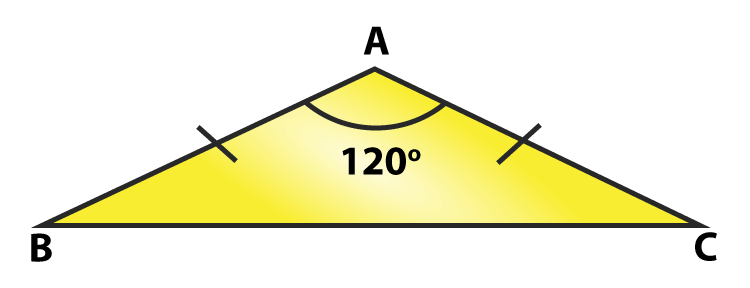To find: ∠ B and ∠ C.

Here, Δ ABC is an isosceles triangle since AB = AC

∠ B = ∠ C ……… (i)

[Angles opposite to equal sides are equal]

We know, sum of angles in a triangle = 180°

∠ A + ∠ B + ∠ C = 180°

∠ A + ∠ B + ∠ B= 180° (using (i)

1200 + 2∠B = 1800

2∠B = 1800 – 1200 = 600

∠ B = 30o

Therefore, ∠ B = ∠ C = 30

Question 6: In a Δ ABC, if AB = AC and ∠ B = 70°, find ∠ A.

Solution:

Given: In a Δ ABC, AB = AC and ∠B = 70°

∠ B = ∠ C [Angles opposite to equal sides are equal]

Therefore, ∠ B = ∠ C = 70

Sum of angles in a triangle = 180∘

∠ A + ∠ B + ∠ C = 180o

∠ A + 70o + 70o = 180o

∠ A = 180o – 140o

∠ A = 40o

### Exercise 10.2

Question 1: In figure, it is given that RT = TS, ∠ 1 = 2 ∠ 2 and ∠4 = 2(∠3). Prove that ΔRBT ≅ ΔSAT.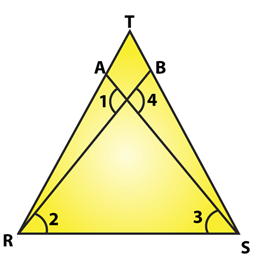Solution:

In the figure,

RT = TS ……(i)

∠ 1 = 2 ∠ 2 ……(ii)

And ∠ 4 = 2 ∠ 3 ……(iii)

To prove: ΔRBT ≅ ΔSAT

Let the point of intersection RB and SA be denoted by O

∠ AOR = ∠ BOS [Vertically opposite angles]

or ∠ 1 = ∠ 4

2 ∠ 2 = 2 ∠ 3 [From (ii) and (iii)]

or ∠ 2 = ∠ 3 ……(iv)

Now in Δ TRS, we have RT = TS

=> Δ TRS is an isosceles triangle

∠ TRS = ∠ TSR ……(v)

But, ∠ TRS = ∠ TRB + ∠ 2 ……(vi)

∠ TSR = ∠ TSA + ∠ 3 ……(vii)

Putting (vi) and (vii) in (v) we get

∠ TRB + ∠ 2 = ∠ TSA + ∠ B

=> ∠ TRB = ∠ TSA [From (iv)]

Consider Δ RBT and Δ SAT

RT = ST [From (i)]

∠ TRB = ∠ TSA [From (iv)]

By ASA criterion of congruence, we have

Δ RBT Δ SAT

Question 2: Two lines AB and CD intersect at O such that BC is equal and parallel to AD. Prove that the lines AB and CD bisect at O.

Solution: Lines AB and CD Intersect at O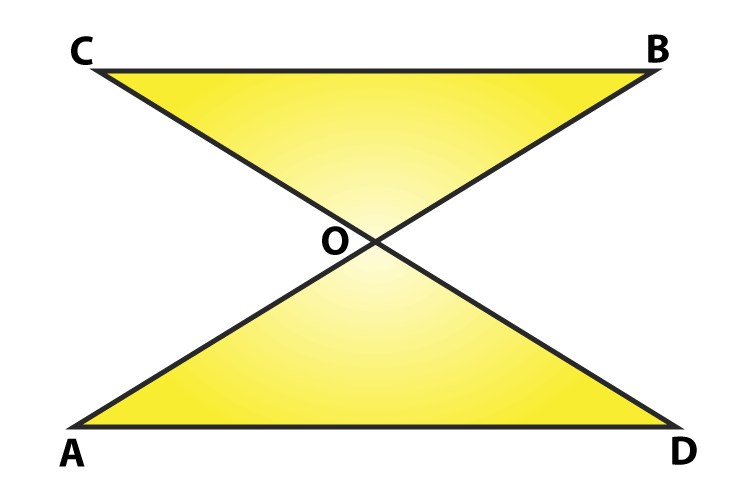Such that BC ∥ AD and

To prove : AB and CD bisect at O.

First we have to prove that Δ AOD ≅ Δ BOC

∠OCB =∠ODA [AD||BC and CD is transversal]

By ASA Criterion:

Δ AOD ≅ Δ BOC

OA = OB and OD = OC (By c.p.c.t.)

Therefore, AB and CD bisect each other at O.

Hence Proved.

Question 3: BD and CE are bisectors of ∠ B and ∠ C of an isosceles Δ ABC with AB = AC. Prove that BD = CE.

Solution:

Δ ABC is isosceles with AB = AC and BD and CE are bisectors of ∠ B and ∠ C We have to prove BD = CE. (Given)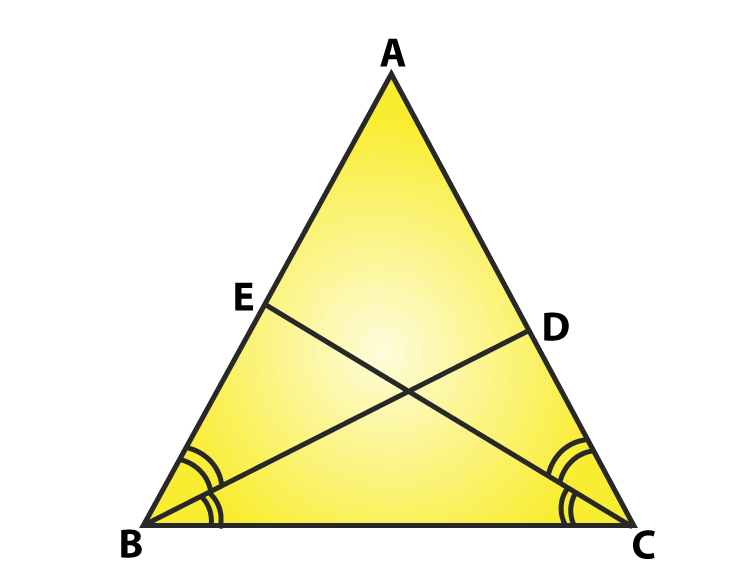Since AB = AC

=> ∠ABC = ∠ACB ……(i)

[Angles opposite to equal sides are equal]

Since BD and CE are bisectors of ∠ B and ∠ C

∠ ABD = ∠ DBC = ∠ BCE = ECA = ∠B/2 = ∠C/2 …(ii)

Now, Consider Δ EBC = Δ DCB

∠ EBC = ∠ DCB [From (i)]

BC = BC [Common side]

∠ BCE = ∠ CBD [From (ii)]

By ASA congruence criterion, Δ EBC ≅ Δ DCB

Since corresponding parts of congruent triangles are equal.

=> CE = BD

or, BD = CE

Hence proved.

### Exercise 10.3

Question 1: In two right triangles one side an acute angle of one are equal to the corresponding side and angle of the other. Prove that the triangles are congruent.

Solution:

In two right triangles one side and acute angle of one are equal to the corresponding side and angles of the other. (Given)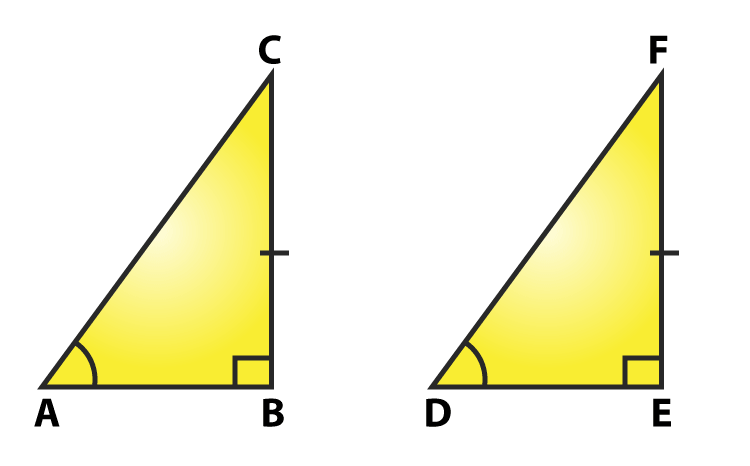To prove: Both the triangles are congruent.

Consider two right triangles such that

∠ B = ∠ E = 90o …….(i)

AB = DE …….(ii)

∠ C = ∠ F ……(iii)

Here we have two right triangles, △ ABC and △ DEF

From (i), (ii) and (iii),

By AAS congruence criterion, we have Δ ABC ≅ Δ DEF

Both the triangles are congruent. Hence proved.

Question 2: If the bisector of the exterior vertical angle of a triangle be parallel to the base. Show that the triangle is isosceles.

Solution:

Let ABC be a triangle such that AD is the angular bisector of exterior vertical angle, ∠EAC and AD ∥ BC.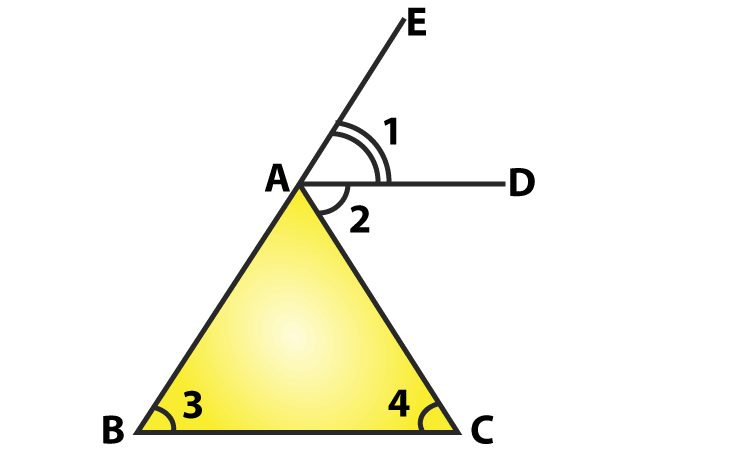From figure,

∠1 = ∠2 [AD is a bisector of ∠ EAC]

∠1 = ∠3 [Corresponding angles]

and ∠2 = ∠4 [alternative angle]

From above, we have ∠3 = ∠4

This implies, AB = AC

Two sides AB and AC are equal.

=> Δ ABC is an isosceles triangle.

Question 3: In an isosceles triangle, if the vertex angle is twice the sum of the base angles, calculate the angles of the triangle.

Solution:

Let Δ ABC be isosceles where AB = AC and ∠ B = ∠ C

Given: Vertex angle A is twice the sum of the base angles B and C. i.e., ∠ A = 2(∠ B + ∠ C)

∠ A = 2(∠ B + ∠ B)

∠ A = 2(2 ∠ B)

∠ A = 4(∠ B)

Now, We know that sum of angles in a triangle =180°

∠ A + ∠ B + ∠ C =180°

4 ∠ B + ∠ B + ∠ B = 180°

6 ∠ B =180°

∠ B = 30°

Since, ∠ B = ∠ C

∠ B = ∠ C = 30°

And ∠ A = 4 ∠ B

∠ A = 4 x 30° = 120°

Therefore, angles of the given triangle are 30° and 30° and 120°.

Question 4: PQR is a triangle in which PQ = PR and is any point on the side PQ. Through S, a line is drawn parallel to QR and intersecting PR at T. Prove that PS = PT.

Solution: Given that PQR is a triangle such that PQ = PR and S is any point on the side PQ and ST ∥ QR.

To prove: PS = PT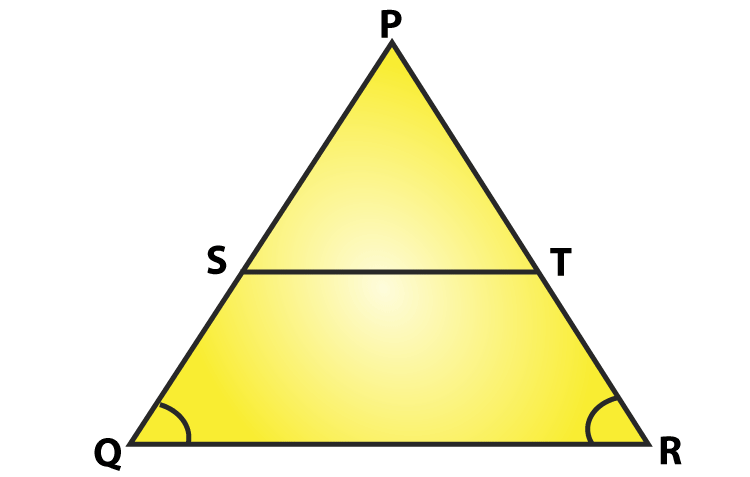Since, PQ= PR, so △PQR is an isosceles triangle.

∠ PQR = ∠ PRQ

Now, ∠ PST = ∠ PQR and ∠ PTS = ∠ PRQ

[Corresponding angles as ST parallel to QR]

Since, ∠ PQR = ∠ PRQ

∠ PST = ∠ PTS

In Δ PST,

∠ PST = ∠ PTS

Δ PST is an isosceles triangle.

Therefore, PS = PT.

Hence proved.

### Exercise 10.4

Question 1: In figure, It is given that AB = CD and AD = BC. Prove that ΔADC ≅ ΔCBA.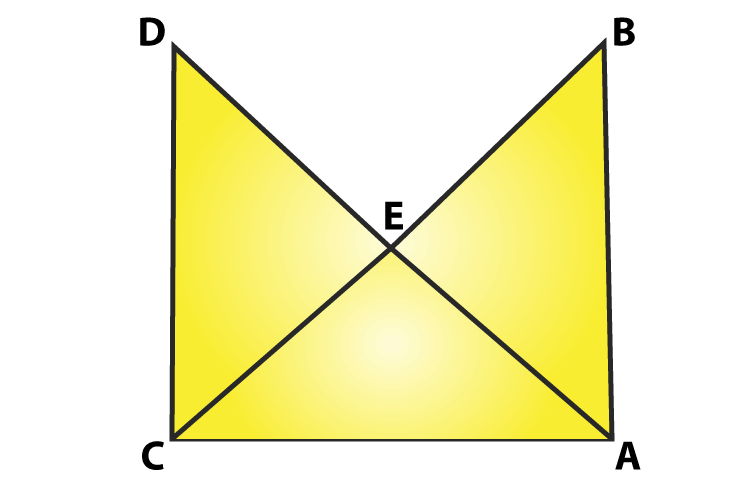Solution:

From figure, AB = CD and AD = BC.

Consider Δ ADC and Δ CBA.

AB = CD [Given]

And AC = AC [Common side]

So, by SSS congruence criterion, we have

Hence proved.

Question 2: In a Δ PQR, if PQ = QR and L, M and N are the mid-points of the sides PQ, QR and RP respectively. Prove that LN = MN.

Solution:

Given: In Δ PQR, PQ = QR and L, M and N are the mid-points of the sides PQ, QR and RP respectively

To prove: LN = MN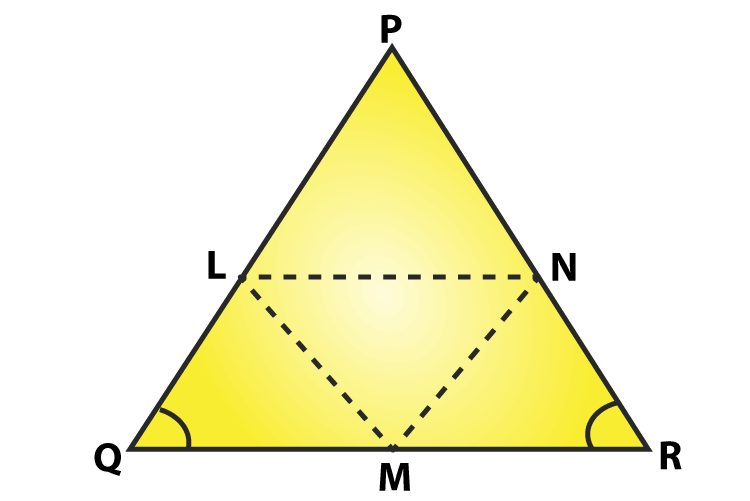Join L and M, M and N, N and L

We have PL = LQ, QM = MR and RN = NP

[Since, L, M and N are mid-points of PQ, QR and RP respectively]

And also PQ = QR

PL = LQ = QM = MR = PN = LR …….(i)

[ Using mid-point theorem]

MN ∥ PQ and MN = PQ/2

MN = PL = LQ ……(ii)

Similarly, we have

LN ∥ QR and LN = (1/2)QR

LN = QM = MR ……(iii)

From equation (i), (ii) and (iii), we have

PL = LQ = QM = MR = MN = LN

This implies, LN = MN

Hence Proved.

### Exercise 10.5

Question 1: ABC is a triangle and D is the mid-point of BC. The perpendiculars from D to AB and AC are equal. Prove that the triangle is isosceles.

Solution:

Given: D is the midpoint of BC and PD = DQ in a triangle ABC.

To prove: ABC is isosceles triangle.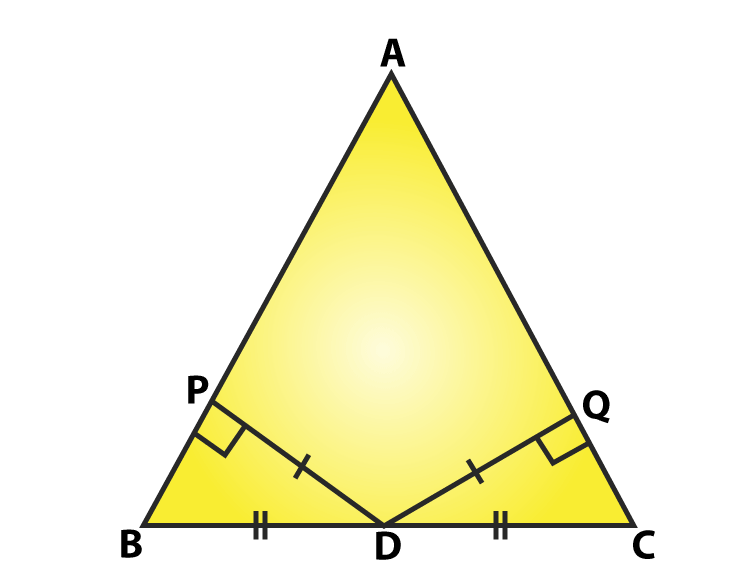In △BDP and △CDQ

PD = QD (Given)

BD = DC (D is mid-point)

∠BPD = ∠CQD = 90o

By RHS Criterion: △BDP ≅ △CDQ

BP = CQ … (i) (By CPCT)

In △APD and △AQD

PD = QD (given)

APD = AQD = 90 o

By RHS Criterion: △APD ≅ △AQD

So, PA = QA … (ii) (By CPCT)

BP + PA = CQ + QA

AB = AC

Two sides of the triangle are equal, so ABC is an isosceles.

Question 2: ABC is a triangle in which BE and CF are, respectively, the perpendiculars to the sides AC and AB. If BE = CF, prove that Δ ABC is isosceles

Solution:

ABC is a triangle in which BE and CF are perpendicular to the sides AC and AS respectively s.t. BE = CF.

To prove: Δ ABC is isosceles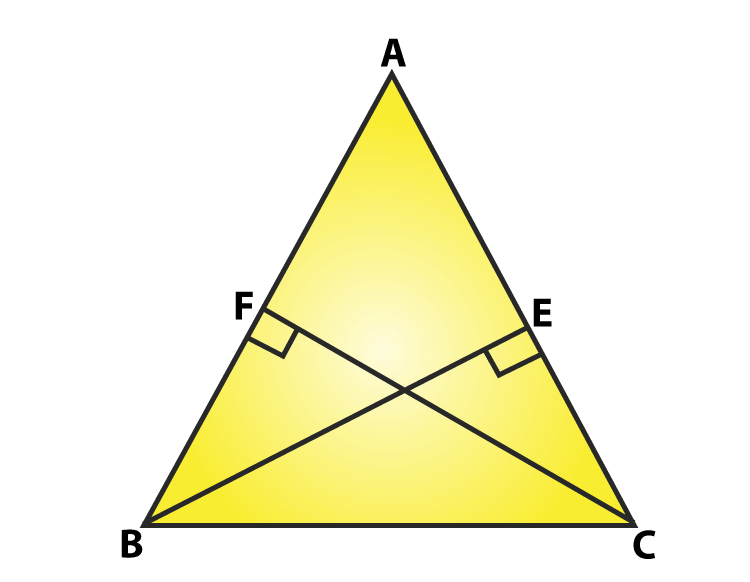In Δ BCF and Δ CBE,

∠ BFC = CEB = 90o [Given]

BC = CB [Common side]

And CF = BE [Given]

By RHS congruence criterion: ΔBFC ≅ ΔCEB

So, ∠ FBC = ∠ EBC [By CPCT]

=>∠ ABC = ∠ ACB

AC = AB [Opposite sides to equal angles are equal in a triangle]

Two sides of triangle ABC are equal.

Therefore, Δ ABC is isosceles. Hence Proved.

Question 3: If perpendiculars from any point within an angle on its arms are congruent. Prove that it lies on the bisector of that angle.

Solution: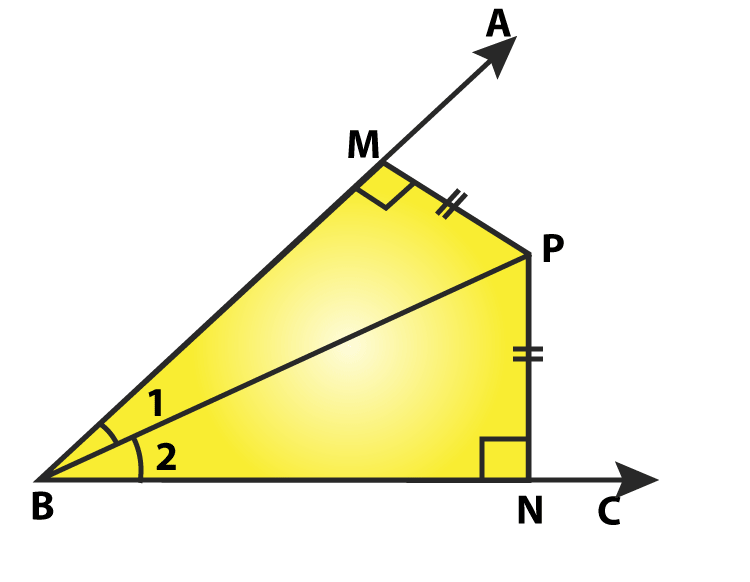Consider an angle ABC and BP be one of the arm within the angle.

Draw perpendiculars PN and PM on the arms BC and BA.

In Δ BPM and Δ BPN,

∠ BMP = ∠ BNP = 90° [given]

BP = BP [Common side]

MP = NP [given]

By RHS congruence criterion: ΔBPM≅ΔBPN

So, ∠ MBP = ∠ NBP [ By CPCT]

BP is the angular bisector of ∠ABC.

Hence proved

### Exercise 10.6 Page No: 10.66

Question 1: In Δ ABC, if ∠ A = 40° and ∠ B = 60°. Determine the longest and shortest sides of the triangle.

Solution: In Δ ABC, ∠ A = 40° and ∠ B = 60°

We know, sum of angles in a triangle = 180°

∠ A + ∠ B + ∠ C = 180°

40° + 60° + ∠ C = 180°

∠ C = 180° – 100° = 80°

∠ C = 80°

Now, 40° < 60° < 80°

=> ∠ A < ∠ B < ∠ C

=> ∠ C is greater angle and ∠ A is smaller angle.

Now, ∠ A < ∠ B < ∠ C

We know, side opposite to greater angle is larger and side opposite to smaller angle is smaller.

Therefore, BC < AC < AB

AB is longest and BC is shortest side.

Question 2: In a Δ ABC, if ∠ B = ∠ C = 45°, which is the longest side?

Solution: In Δ ABC, ∠ B = ∠ C = 45°

Sum of angles in a triangle = 180°

∠ A + ∠ B + ∠ C = 180°

∠ A + 45° + 45° = 180°

∠ A = 180° – (45° + 45°) = 180° – 90° = 90°

∠ A = 90°

=> ∠ B = ∠ C < ∠ A

Therefore, BC is the longest side.

Question 3: In Δ ABC, side AB is produced to D so that BD = BC. If ∠ B = 60° and ∠ A = 70°.

Solution: In Δ ABC, side AB is produced to D so that BD = BC.

∠ B = 60°, and ∠ A = 70°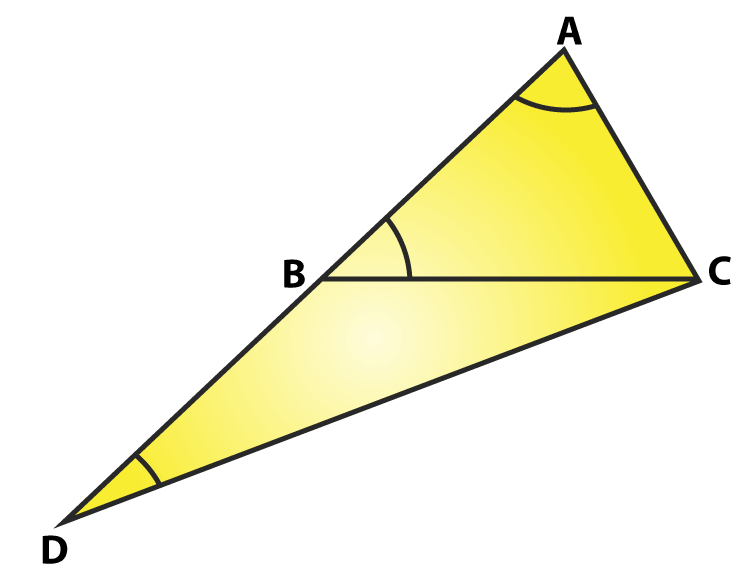Construction: Join C and D

We know, sum of angles in a triangle = 180°

∠ A + ∠ B + ∠ C = 180°

70° + 60° + ∠ C = 180°

∠ C = 180° – (130°) = 50°

∠ C = 50°

∠ ACB = 50° ……(i)

And also in Δ BDC

∠ DBC =180° – ∠ ABC = 180 – 60° = 120°

[∠DBA is a straight line]

and BD = BC [given]

∠ BCD = ∠ BDC [Angles opposite to equal sides are equal]

Sum of angles in a triangle =180°

∠ DBC + ∠ BCD + ∠ BDC = 180°

120° + ∠ BCD + ∠ BCD = 180°

120° + 2∠ BCD = 180°

2∠ BCD = 180° – 120° = 60°

∠ BCD = 30°

∠ BCD = ∠ BDC = 30° ….(ii)

∠ DAC = 70° [given]

∠ ADC = 30° [From (ii)]

∠ ACD = ∠ ACB+ ∠ BCD = 50° + 30° = 80° [From (i) and (ii)]

Now, ∠ ADC < ∠ DAC < ∠ ACD

[Side opposite to greater angle is longer and smaller angle is smaller]

Hence proved.

Question 4: Is it possible to draw a triangle with sides of length 2 cm, 3 cm and 7 cm?

Solution:

Lengths of sides are 2 cm, 3 cm and 7 cm.

A triangle can be drawn only when the sum of any two sides is greater than the third side.

So, let’s check the rule.

2 + 3 ≯ 7 or 2 + 3 < 7

2 + 7 > 3

and 3 + 7 > 2

Here 2 + 3 ≯ 7

So, the triangle does not exit.

### Exercise VSAQs

Question 1: In two congruent triangles ABC and DEF, if AB = DE and BC = EF. Name the pairs of equal angles.

Solution:

In two congruent triangles ABC and DEF, if AB = DE and BC = EF, then

∠A = ∠D, ∠B = ∠ E and ∠ C = ∠F

Question 2: In two triangles ABC and DEF, it is given that ∠A = ∠D, ∠B = ∠ E and ∠ C = ∠F. Are the two triangles necessarily congruent?

Solution:
No.

Reason: Two triangles are not necessarily congruent, because we know only angle-angle-angle (AAA) criterion. This criterion can produce similar but not congruent triangles.

Question 3: If ABC and DEF are two triangles such that AC = 2.5 cm, BC = 5 cm, C = 75o, DE = 2.5 cm, DF = 5 cm and D = 75o. Are two triangles congruent?

Solution: Yes.

Reason: Given triangles are congruent as AC = DE = 2.5 cm, BC = DF = 5 cm and

∠D = ∠C = 75o.

By SAS theorem triangle ABC is congruent to triangle EDF.

Question 4: In two triangles ABC and ADC, if AB = AD and BC = CD. Are they congruent?

Solution: Yes.

Reason: Given triangles are congruent as

BC = CD and

AC [ common side]

By SSS theorem triangle ABC is congruent to triangle ADC.

Question 5: In triangles ABC and CDE, if AC = CE, BC = CD, ∠A = 60o, ∠C = 30 o and ∠D = 90 o. Are two triangles congruent?

Solution: Yes.

Reason: Given triangles are congruent

Here AC = CE

BC = CD

∠B = ∠D = 90°

By SSA criteria triangle ABC is congruent to triangle CDE.

Question 6: ABC is an isosceles triangle in which AB = AC. BE and CF are its two medians. Show that BE = CF.

Solution: ABC is an isosceles triangle (given)

AB = AC (given)

BE and CF are two medians (given)

To prove: BE = CF

In △CFB and △BEC

CE = BF (Since, AC = AB = AC/2 = AB/2 = CE = BF)

BC = BC (Common)

∠ECB = ∠FBC (Angle opposite to equal sides are equal)

By SAS theorem: △CFB ≅ △BEC

So, BE = CF (By c.p.c.t)

## RD Sharma Solutions for Chapter 10 Congruent Triangles

In the 10th chapter of Class 9 RD Sharma Solutions students will study important concepts listed below:

• Congruence of Line segments
• Congruence of Angles
• Congruence of Triangles
• Congruence Relation
• Some inequality Relations in a Triangle

Students can download  RD Sharma Solutions for all classes and chapters start practising to score good marks.